# 3-D flow of a compressible viscous micropolar fluid with spherical symmetry: a local existence theorem

## Abstract

We consider nonstationary 3-D flow of a compressible viscous heat-conducting micropolar fluid in the domain to be the subset of ${\mathbf{R}}^{3}$ bounded with two concentric spheres that present solid thermoinsulated walls. In thermodynamical sense fluid is perfect and polytropic. Assuming that the initial density and temperature are strictly positive we will prove that for smooth enough spherically symmetric initial data there exists a spherically symmetric generalized solution locally in time.

## 1 Introduction

The theory of micropolar fluids is introduced in  by Eringen. Various problems with different initial and boundary conditions for incompressible micropolar fluid are presented in , but the theory of compressible micropolar fluid is still in the beginning. N. Mujakovic in  developed the model for one-dimensional isotropic, viscous, compressible micropolar fluid which is in thermodynamical sense perfect and polytropic. In the same work, the local existence of the solution for homogeneous boundary conditions is proved. N. Mujakovic in  and in the references cited therein proved the local and global existence of inhomogeneous boundary conditions for velocity and microrotation as well as stabilization and regularity. In  the Cauchy problem for the described problem was also considered. In the last years we find some interesting works with different kind of problems concerning micropolar fluid, e.g., [6, 7], but till now the described model of compressible micropolar fluid in three-dimensional case has not been considered.

In this work we consider the three-dimensional model with spherical symmetry. The first article in which the problem of spherical symmetry was described is , but for classical fluid. The spherical symmetry for classical fluid is also considered in articles .

In the setting of the field equations we use the Eulerian description.

In what follows we use the notation:

ρ - mass density

v - velocity

p - pressure

T - stress tensor

${\mathbf{T}}_{x}$ - an axial vector with the Cartesian components ${\left({\mathbf{T}}_{x}\right)}_{i}={\epsilon }_{ijk}{\mathbf{T}}_{jk}$, where ${\epsilon }_{ijk}$ is Levi-Civita alternating tensora

$sym\mathbf{T}=\frac{1}{2}\left(\mathbf{T}+{\mathbf{T}}^{T}\right)$, $skw\mathbf{T}=\frac{1}{2}\left(\mathbf{T}-{\mathbf{T}}^{T}\right)$

ω - microrotation velocity

${\mathbit{\omega }}_{skw}$ - skew tensor with Cartesian components ${\left({\mathbit{\omega }}_{skw}\right)}_{ij}={\epsilon }_{kij}{\mathbit{\omega }}_{k}$

${\mathrm{j}}_{\mathrm{I}}$ - microinertia density (a positive scalar field)

C - couple stress tensor

θ - absolute temperature

E - internal energy density

q - heat flux density vector

f - body force density

g - body couple density

δ - body heat density

The problem we consider here is based on local forms of the conservation laws for mass, momentum, momentum moment and energy, which are stated respectively as follows:

$\stackrel{˙}{\rho }+\rho div\mathbf{v}=0,$
(1)
$\rho \stackrel{˙}{\mathbf{v}}=div\mathbf{T}+\rho \mathbf{f},$
(2)
$\rho {\mathrm{j}}_{\mathrm{I}}\stackrel{˙}{\mathbit{\omega }}=div\mathbf{C}+{\mathbf{T}}_{x}+\rho \mathbf{g},$
(3)
$\rho \stackrel{˙}{E}=\mathbf{T}:\mathrm{\nabla }\mathbf{v}+\mathbf{C}:\mathrm{\nabla }\mathbit{\omega }-{\mathbf{T}}_{x}\cdot \mathbit{\omega }-div\mathbf{q}+\rho \delta ,$
(4)

where $\stackrel{˙}{\mathbf{a}}$ denotes material derivative of a field a:

$\stackrel{˙}{\mathbf{a}}=\frac{\partial \mathbf{a}}{\partial t}+\left(\mathrm{\nabla }\mathbf{a}\right)\cdot \mathbf{v}.$

The scalar product of tensors A and B is defined by

$\mathbf{A}:\mathbf{B}=tr\left({\mathbf{A}}^{T}\mathbf{B}\right).$

The linear constitutive equations for stress tensor, couple stress tensor and heat flux density vector are respectively in the forms:

$\mathbf{T}=\left(-p+\lambda div\mathbf{v}\right)\mathbf{I}+2\mu sym\mathrm{\nabla }\mathbf{v}-2{\mu }_{r}skw\mathrm{\nabla }\mathbf{v}-2{\mu }_{r}{\mathbit{\omega }}_{skw},$
(5)
$\mathbf{C}={c}_{0}\left(div\mathbit{\omega }\right)\mathbf{I}+2{c}_{d}sym\mathrm{\nabla }\mathbit{\omega }-2{c}_{a}skw\mathrm{\nabla }\mathbit{\omega },$
(6)
$\mathbf{q}=-k\mathrm{\nabla }\theta ,$
(7)

where

λ, μ - coefficients of viscosity,

${\mu }_{r}$, ${c}_{0}$, ${c}_{d}$, ${c}_{a}$ - coefficients of microviscosity,

k - heat conduction coefficient

are constants with the properties

$\mu \ge 0,\phantom{\rule{2em}{0ex}}3\lambda +2\mu \ge 0,\phantom{\rule{2em}{0ex}}{\mu }_{r}\ge 0,$
(8)
${c}_{d}\ge 0,\phantom{\rule{2em}{0ex}}3{c}_{0}+2{c}_{d}\ge 0,\phantom{\rule{2em}{0ex}}|{c}_{d}-{c}_{a}|\le {c}_{d}+{c}_{a},\phantom{\rule{2em}{0ex}}k\ge 0.$
(9)

Assuming that the fluid is perfect and polytropic, for pressure and internal energy we have the equations

$p=R\rho \theta ,$
(10)
$E={c}_{v}\theta ,$
(11)

where R and ${c}_{v}$ are positive constants.

Let $\mathrm{\Omega }=\left\{\mathbf{x}\in {\mathbf{R}}^{3},a<|\mathbf{x}|, $a>0$, denote the domain bounded by two concentric spheres with radii a and b. The boundary of the described domain is . We shall consider the problem (1)-(11) in the region ${Q}_{T}=\mathrm{\Omega }×\phantom{\rule{0.2em}{0ex}}\right]0,T\left[$ (where $T>0$ is arbitrary) with the following initial conditions:

$\rho \left(\mathbf{x},0\right)={\rho }_{0}\left(\mathbf{x}\right),\phantom{\rule{2em}{0ex}}\mathbf{v}\left(\mathbf{x},0\right)={\mathbf{v}}_{0}\left(\mathbf{x}\right),\phantom{\rule{2em}{0ex}}\mathbit{\omega }\left(\mathbf{x},0\right)={\mathbit{\omega }}_{\mathbf{0}}\left(\mathbf{x}\right),\phantom{\rule{2em}{0ex}}\theta \left(\mathbf{x},0\right)={\theta }_{0}\left(\mathbf{x}\right),$
(12)

for $\mathbf{x}\in \mathrm{\Omega }$ and boundary conditions

$\mathbf{v}{|}_{\partial \mathrm{\Omega }}=0,\phantom{\rule{2em}{0ex}}\mathbit{\omega }{|}_{\partial \mathrm{\Omega }}=0,\phantom{\rule{2em}{0ex}}\frac{\partial \theta }{\partial \mathbit{\nu }}{|}_{\partial \mathrm{\Omega }}=0,$
(13)

for $0; the vector ν is an exterior unit normal vector.

For simplicity we also assume that $\mathbf{f}=\mathbf{g}=0$ and $\delta =0$.

The initial boundary problems for the system (1)-(13) so far have not been considered in three-dimensional case. The same and similar models in one-dimensional case were considered in [3, 5, 13] and . In  the three-dimensional model was considered but for an incompressible micropolar fluid.

In this paper we prove the local existence of generalized spherically symmetric solution to the problem (1)-(13) in the domain Ω, assuming that the initial functions are also spherically symmetric. In the proof we use the Faedo-Galerkin method. We follow some ideas of  where this method was applied to a classical fluid (where microrotation is equal to zero) in one-dimensional case as well as the ideas from  and  where the same result as here was provided for one-dimensional case.

The paper is organized as follows. In the second section, we derive a spherically symmetric form of (1)-(4), introduce Lagrangian description, and present the main result. In the third section, we consider an approximate problem and get an approximate solution for each $n\in \mathbf{N}$. In the forth section, we prove uniform a priori estimates for the approximate solutions. The proof of the main result is given in the fifth section.

## 2 Spherically symmetric form and the main result

We first derive the spherically symmetric form of (1)-(7) and (10)-(11). A spherically symmetric solution of (1)-(7) has the form:

$\begin{array}{r}{v}_{i}\left(\mathbf{x},t\right)=\frac{{x}_{i}}{r}v\left(r,t\right),\phantom{\rule{2em}{0ex}}{\omega }_{i}\left(\mathbf{x},t\right)=\frac{{x}_{i}}{r}\omega \left(r,t\right),\phantom{\rule{1em}{0ex}}i=1,2,3,\\ \rho \left(\mathbf{x},t\right)=\rho \left(r,t\right),\phantom{\rule{2em}{0ex}}\theta \left(\mathbf{x},t\right)=\theta \left(r,t\right),\end{array}$
(14)

where $\mathbf{x}=\left({x}_{1},{x}_{2},{x}_{3}\right)\in {\mathbf{R}}^{3}$, $r=|\mathbf{x}|$, $\mathbf{v}=\left({v}_{1},{v}_{2},{v}_{3}\right)$ and $\mathbit{\omega }=\left({\omega }_{1},{\omega }_{2},{\omega }_{3}\right)$. We assume that

${\rho }_{0}\left(\mathbf{x}\right)={\rho }_{0}\left(r\right),\phantom{\rule{2em}{0ex}}{\mathbf{v}}_{0}\left(\mathbf{x}\right)=\frac{\mathbf{x}}{r}{v}_{0}\left(r\right),\phantom{\rule{2em}{0ex}}{\mathbit{\omega }}_{0}\left(\mathbf{x}\right)=\frac{\mathbf{x}}{r}{\omega }_{0}\left(r\right),\phantom{\rule{2em}{0ex}}{\theta }_{0}\left(\mathbf{x}\right)={\theta }_{0}\left(r\right),$
(15)

where ${\rho }_{0}$, ${v}_{0}$, ${\omega }_{0}$ and ${\theta }_{0}$ are known real functions defined on $\right]a,b\left[$, and thus we reduce system (1)-(7) and conditions (10)-(13) to the following equations for $\rho \left(r,t\right)$, $v\left(r,t\right)$, $\omega \left(r,t\right)$ and $\theta \left(r,t\right)$ of the form:

$\frac{\partial \rho }{\partial t}+\frac{\partial }{\partial r}\left(v\rho \right)+\frac{2\rho }{r}v=0,$
(16)
$\rho \left(\frac{\partial v}{\partial t}+v\frac{\partial v}{\partial r}\right)=-R\frac{\partial }{\partial r}\left(\rho \theta \right)+\left(\lambda +2\mu \right)\frac{\partial }{\partial r}\left(\frac{\partial v}{\partial r}+2\frac{v}{r}\right),$
(17)
$\rho {\mathrm{j}}_{\mathrm{I}}\left(\frac{\partial \omega }{\partial t}+v\frac{\partial \omega }{\partial r}\right)=-4{\mu }_{r}\omega +\left({c}_{0}+2{c}_{d}\right)\frac{\partial }{\partial r}\left(\frac{\partial \omega }{\partial r}+2\frac{\omega }{r}\right),$
(18)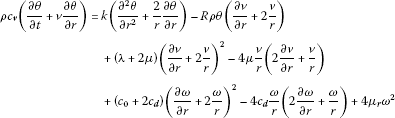(19)

with the following initial and boundary conditions

$\begin{array}{r}\rho \left(r,0\right)={\rho }_{0}\left(r\right),\phantom{\rule{2em}{0ex}}v\left(r,0\right)={v}_{0}\left(r\right),\phantom{\rule{2em}{0ex}}\omega \left(r,0\right)={\omega }_{0}\left(r\right),\\ \theta \left(r,0\right)={\theta }_{0}\left(r\right),\phantom{\rule{1em}{0ex}}r\in \phantom{\rule{0.2em}{0ex}}\right]a,b\left[,\end{array}$
(20)
$\begin{array}{r}v\left(a,t\right)=v\left(b,t\right)=0,\phantom{\rule{2em}{0ex}}\omega \left(a,t\right)=\omega \left(b,t\right)=0,\\ \frac{\partial \theta }{\partial r}\left(a,t\right)=\frac{\partial \theta }{\partial r}\left(b,t\right)=0,\phantom{\rule{1em}{0ex}}0
(21)

To investigate the local existence, it is convenient to transform the system (16)-(19) to that in Lagrangian coordinates. The Eulerian coordinates $\left(r,t\right)$ are connected to the Lagrangian coordinates $\left(\xi ,t\right)$ by the relation

$r\left(\xi ,t\right)={r}_{0}\left(\xi \right)+{\int }_{0}^{t}\stackrel{˜}{v}\left(\xi ,t\right)\phantom{\rule{0.2em}{0ex}}d\tau ,\phantom{\rule{2em}{0ex}}{r}_{0}\left(\xi \right)=r\left(\xi ,0\right)=\xi ,$
(22)

where $\stackrel{˜}{v}\left(\xi ,t\right)$ is defined by

$\stackrel{˜}{v}\left(\xi ,t\right)=v\left(r\left(\xi ,t\right),t\right).$
(23)

We introduce the new function η by

$\eta \left(\xi \right)={\int }_{a}^{\xi }{s}^{2}{\rho }_{0}\left(s\right)\phantom{\rule{0.2em}{0ex}}ds.$
(24)

Note that if ${\rho }_{0}\left(s\right)>0$ for $s\in \phantom{\rule{0.2em}{0ex}}\right]a,b\left[$ (which is assumed in Theorem 2.1 later), then there exists an inverse function ${\eta }^{-1}$. Let the constant L be defined as

$\eta \left(b\right)={\int }_{a}^{b}{s}^{2}{\rho }_{0}\left(s\right)\phantom{\rule{0.2em}{0ex}}ds=L.$
(25)

From (16) we can easily get the equation

$\rho \frac{\partial r}{\partial \xi }{r}^{2}={\rho }_{0}\left(\xi \right){\xi }^{2},$
(26)

i.e.,

${\int }_{a}^{r\left(\xi ,t\right)}\rho \left(s,t\right){s}^{2}\phantom{\rule{0.2em}{0ex}}ds={\int }_{a}^{\xi }{\rho }_{0}\left(s\right){s}^{2}\phantom{\rule{0.2em}{0ex}}ds.$
(27)

It is useful to introduce the next coordinate

${x}^{\prime }={L}^{-1}\eta \left(\xi \right)$
(28)

and the following functions

$\begin{array}{r}{\rho }^{\prime }\left({x}^{\prime },t\right)=\stackrel{˜}{\rho }\left({\eta }^{-1}\left({x}^{\prime }L\right),t\right),\\ {v}^{\prime }\left({x}^{\prime },t\right)=\stackrel{˜}{v}\left({\eta }^{-1}\left({x}^{\prime }L\right),t\right),\\ {\omega }^{\prime }\left({x}^{\prime },t\right)=\stackrel{˜}{\omega }\left({\eta }^{-1}\left({x}^{\prime }L\right),t\right),\\ {\theta }^{\prime }\left({x}^{\prime },t\right)=\stackrel{˜}{\theta }\left({\eta }^{-1}\left({x}^{\prime }L\right),t\right),\\ {r}^{\prime }\left({x}^{\prime },t\right)=r\left({\eta }^{-1}\left({x}^{\prime }L\right),t\right),\\ {\rho }_{0}^{\prime }\left({x}^{\prime }\right)={\rho }_{0}\left({\eta }^{-1}\left({x}^{\prime }L\right)\right),\\ {v}_{0}^{\prime }\left({x}^{\prime }\right)={v}_{0}\left({\eta }^{-1}\left({x}^{\prime }L\right)\right),\\ {\omega }_{0}^{\prime }\left({x}^{\prime }\right)={\omega }_{0}\left({\eta }^{-1}\left({x}^{\prime }L\right)\right),\\ {\theta }_{0}^{\prime }\left({x}^{\prime }\right)={\theta }_{0}\left({\eta }^{-1}\left({x}^{\prime }L\right)\right),\\ {r}_{0}^{\prime }\left({x}^{\prime }\right)={r}_{0}\left({\eta }^{-1}\left({x}^{\prime }L\right)\right)={\eta }^{-1}\left({x}^{\prime }L\right).\end{array}$

Similarly as in , for a new coordinate ${x}^{\prime }$ we get

$0={L}^{-1}\eta \left(a\right)\le {x}^{\prime }\le {L}^{-1}\eta \left(b\right)=1.$
(29)

Taking into account (26) and (24), we obtain that the functions ${\rho }^{\prime }$, ${v}^{\prime }$, ${\omega }^{\prime }$, ${\theta }^{\prime }$ and ${r}^{\prime }$ satisfy the system that we write omitting the primes for simplicity:

$\frac{\partial \rho }{\partial t}=-\frac{1}{L}{\rho }^{2}\frac{\partial }{\partial x}\left({r}^{2}v\right),$
(30)
$\frac{\partial v}{\partial t}=-\frac{R}{L}{r}^{2}\frac{\partial }{\partial x}\left(\rho \theta \right)+\frac{\lambda +2\mu }{{L}^{2}}{r}^{2}\frac{\partial }{\partial x}\left(\rho \frac{\partial }{\partial x}\left({r}^{2}v\right)\right),$
(31)
$\rho \frac{\partial \omega }{\partial t}=-\frac{4{\mu }_{r}}{{\mathrm{j}}_{\mathrm{I}}}\omega +\frac{{c}_{0}+2{c}_{d}}{{\mathrm{j}}_{\mathrm{I}}{L}^{2}}{r}^{2}\rho \frac{\partial }{\partial x}\left(\rho \frac{\partial }{\partial x}\left({r}^{2}\omega \right)\right),$
(32)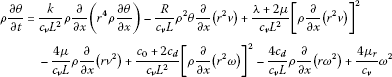(33)

in $\right]0,1\left[\phantom{\rule{0.2em}{0ex}}×\phantom{\rule{0.2em}{0ex}}\right]0,T\left[$, where $T>0$ is arbitrary. Now we have the following boundary and initial conditions

$v\left(0,t\right)=v\left(1,t\right)=0,$
(34)
$\omega \left(0,t\right)=\omega \left(1,t\right)=0,$
(35)
$\frac{\partial \theta }{\partial x}\left(0,t\right)=\frac{\partial \theta }{\partial x}\left(1,t\right)=0,$
(36)

for $t\in \phantom{\rule{0.2em}{0ex}}\right]0,T\left[$,

$\rho \left(x,0\right)={\rho }_{0}\left(x\right),\phantom{\rule{2em}{0ex}}v\left(x,0\right)={v}_{0}\left(x\right),$
(37)
$\omega \left(x,0\right)={\omega }_{0}\left(x\right),\phantom{\rule{2em}{0ex}}\theta \left(x,0\right)={\theta }_{0}\left(x\right),$
(38)

for $x\in \phantom{\rule{0.2em}{0ex}}\right]0,1\left[$. We also have

$r\left(x,t\right)={r}_{0}\left(x\right)+{\int }_{0}^{t}v\left(x,\tau \right)\phantom{\rule{0.2em}{0ex}}d\tau ,\phantom{\rule{1em}{0ex}}\left(x,t\right)\in \phantom{\rule{0.2em}{0ex}}\right]0,1\left[\phantom{\rule{0.2em}{0ex}}×\phantom{\rule{0.2em}{0ex}}\right]0,T\left[.$
(39)

From

$\frac{\partial r}{\partial x}\left(x,t\right)=\frac{L}{\rho \left(x,t\right){r}^{2}\left(x,t\right)}$

putting $t=0$ and integrating over $\right]0,x\left[$, we get

${r}_{0}\left(x\right)={\left({a}^{3}+3L{\int }_{0}^{x}\frac{1}{{\rho }_{0}\left(y\right)}\phantom{\rule{0.2em}{0ex}}dy\right)}^{\frac{1}{3}},\phantom{\rule{1em}{0ex}}x\in \phantom{\rule{0.2em}{0ex}}\right]0,1\left[,$
(40)

where $a>0$ is a radius of the smaller boundary sphere.

We assume the inequalities

(41)

where $m\in {\mathbf{R}}^{+}$.

Before stating the main result, we introduce the following definition.

Definition 2.1 A generalized solution of the problem (30)-(38) in the domain ${Q}_{T}=\phantom{\rule{0.2em}{0ex}}\right]0,1\left[\phantom{\rule{0.2em}{0ex}}×\phantom{\rule{0.2em}{0ex}}\right]0,T\left[$ is a function

$\left(x,t\right)↦\left(\rho ,v,\omega ,\theta \right)\left(x,t\right),\phantom{\rule{1em}{0ex}}\left(x,t\right)\in {Q}_{T},$
(42)

where

$\rho \in {L}^{\mathrm{\infty }}\left(0,T;{H}^{1}\left(\right]0,1\left[\right)\right)\cap {H}^{1}\left({Q}_{T}\right),\phantom{\rule{1em}{0ex}}\underset{{Q}_{T}}{inf}\rho >0,$
(43)
$v,\omega ,\theta \in {L}^{\mathrm{\infty }}\left(0,T;{H}^{1}\left(\right]0,1\left[\right)\right)\cap {H}^{1}\left({Q}_{T}\right)\cap {L}^{2}\left(0,T;{H}^{2}\left(\right]0,1\left[\right)\right),$
(44)

that satisfies Equations (30)-(33) a.e. in ${Q}_{T}$ and conditions (34)-(38) in the sense of traces.

Remark 2.1 From the embedding and interpolation theorems (e.g.,  and ) one can conclude that from (43) and (44) it follows:

$\rho \in {L}^{\mathrm{\infty }}\left(0,T;C\left(\left[0,1\right]\right)\right)\cap C\left(\left[0,T\right],{L}^{2}\left(\right]0,1\left[\right)\right),$
(45)
$v,\omega ,\theta \in {L}^{2}\left(0,T;{C}^{\left(1\right)}\left(\left[0,1\right]\right)\right)\cap C\left(\left[0,T\right],{H}^{1}\left(\right]0,1\left[\right)\right),$
(46)
$v,\omega ,\theta \in C\left({\overline{Q}}_{T}\right).$
(47)

It is easy to check that the solution (42) with properties (43)-(44) satisfies the condition for a strong solution of the described problem.

The aim of this paper is to prove the following statements.

Theorem 2.1 Let the functions

${\rho }_{0},{\theta }_{0}\in {H}^{1}\left(\right]0,1\left[\right),$
(48)
${v}_{0},{\omega }_{0}\in {H}_{0}^{1}\left(\right]0,1\left[\right)$
(49)

satisfy conditions (41). Then there exists ${T}_{0}$, $0<{T}_{0}\le T$, such that the problem (30)-(38) has a generalized solution in ${Q}_{0}={Q}_{{T}_{0}}$, having the property

(50)

For the function r, it holds

$r\in {L}^{\mathrm{\infty }}\left(0,T;{H}^{2}\left(\right]0,1\left[\right)\right)\cap {H}^{2}\left({Q}_{0}\right)\cap C\left({\overline{Q}}_{0}\right),$
(51)
(52)

Remark 2.2 Notice that the function ${r}_{0}$ introduced by (40) belongs to ${H}^{2}\left(\right]0,1\left[\right)$. Because of the embedding ${H}^{1}\left(\right]0,1\left[\right)\subset C\left(\left[0,1\right]\right)$ we can conclude that there exists $M\in {\mathbf{R}}^{+}$ such that

${\rho }_{0}\left(x\right),{v}_{0}\left(x\right),{\omega }_{0}\left(x\right),{\theta }_{0}\left(x\right)\le M,\phantom{\rule{1em}{0ex}}x\in \left[0,1\right].$
(53)

From (40) and (41) we get

${r}_{0}\in {C}^{\left(1\right)}\left(\left[0,1\right]\right),$
(54)
$0
(55)

where ${a}_{1}=L{M}^{-3}$ and ${M}_{1}=L{\left(m{a}^{2}\right)}^{-1}$.

The proof of Theorem 2.1 is essentially based on a careful examination of a priori estimates and a limit procedure. We first study, for each $n\in \mathbf{N}$, an approximate problem and derive the a priori estimates for approximate solutions independent of n by utilizing a technique of Kazhikov [14, 18] and Mujakovic [3, 13] for one-dimensional case. Using the obtained a priori estimates and results of weak compactness, we extract the subsequence of approximate solutions, which, when n tends to infinity, has limit in the same weak sense on $\right]0,1\left[\phantom{\rule{0.2em}{0ex}}×\phantom{\rule{0.2em}{0ex}}\right]0,{T}_{0}\left[$ for sufficiently small ${T}_{0}$, $0<{T}_{0}\le T$. Finally, we show that this limit is the solution to our problem.

## 3 Approximate solutions

We shall find a local generalized solution to the problem (30)-(38) as a limit of approximate solutions

$\left({\rho }^{n},{v}^{n},{\omega }^{n},{\theta }^{n}\right),\phantom{\rule{1em}{0ex}}n\in \mathbf{N},$
(56)

obtained in what follows. First, we introduce the approximations ${v}^{n}$ and ${r}^{n}$ of the functions v and r by

${v}^{n}\left(x,t\right)=\sum _{i=1}^{n}{v}_{i}^{n}\left(t\right)sin\left(\pi ix\right),$
(57)
${r}^{n}\left(x,t\right)={r}_{0}\left(x\right)+{\int }_{0}^{t}{v}^{n}\left(x,\tau \right)\phantom{\rule{0.2em}{0ex}}d\tau ,$
(58)

where ${r}_{0}\left(x\right)$ is defined by (40) and ${v}_{i}^{n}$, $i=1,2,\dots ,n$ are unknown smooth functions defined on an interval $\left[0,{T}_{n}\right]$, ${T}_{n}\le T$.

Then, we can write the solution ${\rho }^{n}$ to the problem

$\frac{\partial {\rho }^{n}}{\partial t}+{L}^{-1}{\left({\rho }^{n}\right)}^{2}\frac{\partial }{\partial x}\left({\left({r}^{n}\right)}^{2}{v}^{n}\right)=0,\phantom{\rule{2em}{0ex}}{\rho }^{n}\left(x,0\right)={\rho }_{0}\left(x\right),$
(59)

in the similar way as in  and  in the form

${\rho }^{n}\left(x,t\right)=\frac{L{\rho }_{0}\left(x\right)}{L+{\rho }_{0}\left(x\right)\frac{\partial }{\partial x}{\int }_{0}^{t}{\left({r}^{n}\right)}^{2}{v}^{n}\phantom{\rule{0.2em}{0ex}}d\tau }.$
(60)

Since ${r}^{n}$ and ${v}^{n}$ are sufficiently smooth functions, we can conclude that the function ${\rho }^{n}$ is continuous on the rectangle $\left[0,1\right]×\left[0,{T}_{n}\right]$ with the property ${\rho }^{n}\left(x,0\right)={\rho }_{0}\left(x\right)\ge m>0$. Because of aforementioned, we can conclude that there exists such ${T}_{n}$, $0<{T}_{n}\le T$ that

(61)

We also introduce the approximations ${\omega }^{n}$ and ${\theta }^{n}$ of the functions ω and θ respectively by

${\omega }^{n}\left(x,t\right)=\sum _{j=1}^{n}{\omega }_{j}^{n}\left(t\right)sin\left(\pi jx\right),$
(62)
${\theta }^{n}\left(x,t\right)=\sum _{k=0}^{n}{\theta }_{k}^{n}\left(t\right)cos\left(\pi kx\right);$
(63)

where ${\omega }_{j}^{n}$ and ${\theta }_{k}^{n}$ are again unknown smooth functions defined on an interval $\left[0,{T}_{n}\right]$, ${T}_{n}\le T$.

Evidently, the boundary conditions

${v}^{n}\left(0,t\right)={v}^{n}\left(1,t\right)={\omega }^{n}\left(0,t\right)={\omega }^{n}\left(1,t\right)=\frac{\partial {\theta }^{n}}{\partial x}\left(0,t\right)=\frac{\partial {\theta }^{n}}{\partial x}\left(1,t\right)=0$
(64)

for $t\in \phantom{\rule{0.2em}{0ex}}\right]0,{T}_{n}\left[$ are satisfied.

According to the Faedo-Galerkin method, we take the following approximate conditions:

$\begin{array}{r}{\int }_{0}^{1}\left[\frac{\partial {v}^{n}}{\partial t}+\frac{R}{L}{\left({r}^{n}\right)}^{2}\frac{\partial }{\partial x}\left({\rho }^{n}{\theta }^{n}\right)\\ \phantom{\rule{1em}{0ex}}-\frac{\lambda +2\mu }{{L}^{2}}{\left({r}^{n}\right)}^{2}\frac{\partial }{\partial x}\left({\rho }^{n}\frac{\partial }{\partial x}\left({\left({r}^{n}\right)}^{2}{v}^{n}\right)\right)\right]sin\left(\pi ix\right)\phantom{\rule{0.2em}{0ex}}dx=0,\end{array}$
(65)
$\begin{array}{r}{\int }_{0}^{1}\left[\frac{\partial {\omega }^{n}}{\partial t}+\frac{4{\mu }_{r}}{{\mathrm{j}}_{\mathrm{I}}}\frac{{\omega }^{n}}{{\rho }^{n}}\\ \phantom{\rule{1em}{0ex}}-\frac{{c}_{0}+2{c}_{d}}{{\mathrm{j}}_{\mathrm{I}}{L}^{2}}{\left({r}^{n}\right)}^{2}\frac{\partial }{\partial x}\left({\rho }^{n}\frac{\partial }{\partial x}\left({\left({r}^{n}\right)}^{2}{\omega }^{n}\right)\right)\right]sin\left(\pi jx\right)\phantom{\rule{0.2em}{0ex}}dx=0,\end{array}$
(66)
$\begin{array}{r}{\int }_{0}^{1}\left[\frac{\partial {\theta }^{n}}{\partial t}-\frac{k}{{c}_{v}{L}^{2}}\frac{\partial }{\partial x}\left({\left({r}^{n}\right)}^{4}{\rho }^{n}\frac{\partial {\theta }^{n}}{\partial x}\right)+\frac{R}{{c}_{v}L}{\rho }^{n}{\theta }^{n}\frac{\partial }{\partial x}\left({\left({r}^{n}\right)}^{2}{v}^{n}\right)\\ \phantom{\rule{1em}{0ex}}-\frac{\lambda +2\mu }{{c}_{v}{L}^{2}}{\rho }^{n}{\left[\frac{\partial }{\partial x}\left({\left({r}^{n}\right)}^{2}{v}^{n}\right)\right]}^{2}+\frac{4\mu }{{c}_{v}L}\frac{\partial }{\partial x}\left({r}^{n}{\left({v}^{n}\right)}^{2}\right)\\ \phantom{\rule{1em}{0ex}}-\frac{{c}_{0}+2{c}_{d}}{{c}_{v}{L}^{2}}{\rho }^{n}{\left[\frac{\partial }{\partial x}\left({\left({r}^{n}\right)}^{2}{\omega }^{n}\right)\right]}^{2}+\frac{4{c}_{d}}{{c}_{v}L}\frac{\partial }{\partial x}\left({r}^{n}{\left({\omega }^{n}\right)}^{2}\right)\\ \phantom{\rule{1em}{0ex}}-\frac{4{\mu }_{r}}{{c}_{v}}\frac{{\left({\omega }^{n}\right)}^{2}}{{\rho }^{n}}\right]cos\left(\pi kx\right)\phantom{\rule{0.2em}{0ex}}dx=0\end{array}$
(67)

for $i,j=1,\dots ,n$, $k=0,1,\dots ,n$.

Let ${v}_{0i}$, ${\omega }_{0j}$, and ${\theta }_{0k}$ be the Fourier coefficients of the functions ${v}_{0}$, ${\omega }_{0}$, and ${\theta }_{0}$ respectively:

$\begin{array}{r}{v}_{0i}=2{\int }_{0}^{1}{v}_{0}\left(x\right)sin\left(\pi ix\right)\phantom{\rule{0.2em}{0ex}}dx,\phantom{\rule{1em}{0ex}}i=1,\dots ,n,\\ {\omega }_{0j}=2{\int }_{0}^{1}{\omega }_{0}\left(x\right)sin\left(\pi jx\right)\phantom{\rule{0.2em}{0ex}}dx,\phantom{\rule{1em}{0ex}}j=1,\dots ,n,\\ {\theta }_{00}={\int }_{0}^{1}{\theta }_{0}\left(x\right)\phantom{\rule{0.2em}{0ex}}dx,\phantom{\rule{2em}{0ex}}{\theta }_{0k}=2{\int }_{0}^{1}{\theta }_{0}\left(x\right)cos\left(\pi kx\right)\phantom{\rule{0.2em}{0ex}}dx,\phantom{\rule{1em}{0ex}}k=1,\dots ,n.\end{array}$

Let ${v}_{0}^{n}$, ${\omega }_{0}^{n}$ and ${\theta }_{0}^{n}$ be

${v}_{0}^{n}\left(x\right)=\sum _{i=1}^{n}{v}_{0i}sin\left(\pi ix\right),$
(68)
${\omega }_{0}^{n}\left(x\right)=\sum _{j=1}^{n}{\omega }_{0j}sin\left(\pi jx\right),$
(69)
${\theta }_{0}^{n}\left(x\right)=\sum _{k=0}^{n}{\theta }_{0k}cos\left(\pi kx\right).$
(70)

We take the initial conditions for ${v}^{n}$, ${\omega }^{n}$ and ${\theta }^{n}$ in the form

${v}^{n}\left(x,0\right)={v}_{0}^{n}\left(x\right),$
(71)
${\omega }^{n}\left(x,0\right)={\omega }_{0}^{n}\left(x\right),$
(72)
${\theta }^{n}\left(x,0\right)={\theta }_{0}^{n}\left(x\right).$
(73)

Let ${z}_{m}^{n}$, ${\lambda }_{pq}^{n}$ and ${\mu }_{slg}^{n}$ be

${z}_{m}^{n}\left(t\right)={\int }_{0}^{t}{v}_{m}^{n}\left(\tau \right)\phantom{\rule{0.2em}{0ex}}d\tau ,\phantom{\rule{1em}{0ex}}m=1,\dots ,n,$
(74)
${\lambda }_{pq}^{n}\left(t\right)={\int }_{0}^{t}{z}_{p}^{n}\left(\tau \right){v}_{q}^{n}\left(\tau \right)\phantom{\rule{0.2em}{0ex}}d\tau ,\phantom{\rule{1em}{0ex}}p,q=1,\dots ,n,$
(75)
${\mu }_{slg}^{n}\left(t\right)={\int }_{0}^{t}{z}_{l}^{n}\left(\tau \right){z}_{s}^{n}\left(\tau \right){v}_{g}^{n}\left(\tau \right)\phantom{\rule{0.2em}{0ex}}d\tau ,\phantom{\rule{1em}{0ex}}s,l,g=1,\dots ,n$
(76)

then we have

${r}^{n}\left(x,t\right)={r}_{0}\left(x\right)+\sum _{m=1}^{n}{z}_{m}^{n}\left(t\right)sin\left(\pi mx\right),$
(77)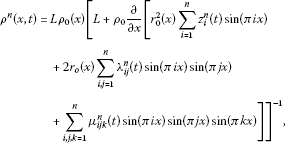(78)

where ${r}_{0}\left(x\right)$ and ${\rho }_{0}\left(x\right)$ are known functions. Taking into account (57), (62), (63), (74)-(78), from (65)-(67) we obtain for $\left\{\left({v}_{i}^{n},{\omega }_{j}^{n},{\theta }_{k}^{n},{z}_{m}^{n},{\lambda }_{pq}^{n},{\mu }_{slg}^{n}\right):i,j,m,p,q,s,l,g=1,\dots ,n,k=0,1,\dots ,n\right\}$, the following Cauchy problem: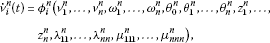(79)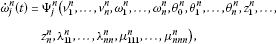(80)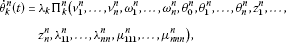(81)
${\stackrel{˙}{z}}_{m}^{n}\left(t\right)={v}_{m}^{n},$
(82)
${\stackrel{˙}{\lambda }}_{pq}^{n}\left(t\right)={z}_{p}^{n}\cdot {v}_{q}^{n},$
(83)
${\stackrel{˙}{\mu }}_{slg}^{n}\left(t\right)={z}_{s}^{n}\cdot {z}_{l}^{n}\cdot {v}_{g}^{n},$
(84)
${v}_{i}^{n}\left(0\right)={v}_{0i},\phantom{\rule{2em}{0ex}}{\omega }_{j}^{n}\left(0\right)={\omega }_{0j},\phantom{\rule{2em}{0ex}}{\theta }_{k}^{n}\left(0\right)={\theta }_{0k},$
(85)
${z}_{m}^{n}\left(0\right)=0,\phantom{\rule{2em}{0ex}}{\lambda }_{pq}^{n}\left(0\right)=0,\phantom{\rule{2em}{0ex}}{\mu }_{slg}^{n}\left(0\right)=0.$
(86)

Here we have ${\lambda }_{0}=1$, ${\lambda }_{k}=2$ for $k=1,2,\dots ,n$ and

$\begin{array}{rl}{\mathrm{\Phi }}_{i}^{n}=& 2{\int }_{0}^{1}\left[\frac{\lambda +2\mu }{{L}^{2}}{\left({r}^{n}\right)}^{2}\frac{\partial }{\partial x}\left({\rho }^{n}\frac{\partial }{\partial x}\left({\left({r}^{n}\right)}^{2}{v}^{n}\right)\right)\\ -\frac{R}{L}{\left({r}^{n}\right)}^{2}\frac{\partial }{\partial x}\left({\rho }^{n}{\theta }^{n}\right)\right]sin\left(\pi ix\right)\phantom{\rule{0.2em}{0ex}}dx,\end{array}$
(87)
$\begin{array}{rl}{\mathrm{\Psi }}_{j}^{n}=& 2{\int }_{0}^{1}\left[\frac{{c}_{0}+2{c}_{d}}{{\mathrm{j}}_{\mathrm{I}}{L}^{2}}{\left({r}^{n}\right)}^{2}\frac{\partial }{\partial x}\left({\rho }^{n}\frac{\partial }{\partial x}\left({\left({r}^{n}\right)}^{2}{\omega }^{n}\right)\right)\\ -\frac{4{\mu }_{r}}{{\mathrm{j}}_{\mathrm{I}}}\frac{{\omega }^{n}}{{\rho }^{n}}\right]sin\left(\pi jx\right)\phantom{\rule{0.2em}{0ex}}dx,\end{array}$
(88)
$\begin{array}{rl}{\mathrm{\Pi }}_{k}^{n}=& {\int }_{0}^{1}\left[\frac{k}{{c}_{v}{L}^{2}}\frac{\partial }{\partial x}\left({\left({r}^{n}\right)}^{4}{\rho }^{n}\frac{\partial {\theta }^{n}}{\partial x}\right)-\frac{R}{{c}_{v}L}{\rho }^{n}{\theta }^{n}\frac{\partial }{\partial x}\left({\left({r}^{n}\right)}^{2}{v}^{n}\right)\\ +\frac{\lambda +2\mu }{{c}_{v}{L}^{2}}{\rho }^{n}{\left[\frac{\partial }{\partial x}\left({\left({r}^{n}\right)}^{2}{v}^{n}\right)\right]}^{2}-\frac{4\mu }{{c}_{v}L}\frac{\partial }{\partial x}\left({r}^{n}{\left({v}^{n}\right)}^{2}\right)\\ +\frac{{c}_{0}+2{c}_{d}}{{c}_{v}{L}^{2}}{\rho }^{n}{\left[\frac{\partial }{\partial x}\left({\left({r}^{n}\right)}^{2}{\omega }^{n}\right)\right]}^{2}-\frac{4{c}_{d}}{{c}_{v}L}\frac{\partial }{\partial x}\left({r}^{n}{\left({\omega }^{n}\right)}^{2}\right)\\ +\frac{4{\mu }_{r}}{{c}_{v}}\frac{{\left({\omega }^{n}\right)}^{2}}{{\rho }^{n}}\right]cos\left(\pi kx\right)\phantom{\rule{0.2em}{0ex}}dx.\end{array}$
(89)

Notice that the functions on the right-hand side of (79)-(84) satisfy the conditions of the Cauchy-Picard theorem [19, 20] and we can easily conclude that the following statements are valid.

Lemma 3.1 For each $n\in N$ there exists such ${T}_{n}$, $0<{T}_{n}\le T$ that the Cauchy problem (79)-(86) has a unique solution defined on $\left[0,{T}_{n}\right]$. The functions ${v}^{n}$, ${\omega }^{n}$ and ${\theta }^{n}$ defined by the formulas (57), (62) and (63) belong to the class ${C}^{\mathrm{\infty }}\left({\overline{Q}}_{n}\right)$, ${Q}_{n}=\phantom{\rule{0.2em}{0ex}}\right]0,1\left[\phantom{\rule{0.2em}{0ex}}×\phantom{\rule{0.2em}{0ex}}\right]0,{T}_{n}\left[$ and satisfy conditions (71)-(73).

From (77) and (78) we can also easily conclude that

${\rho }^{n}\in C\left({\overline{Q}}_{n}\right),$
(90)
${r}^{n}\in {C}^{\left(1\right)}\left({\overline{Q}}_{n}\right).$
(91)

Lemma 3.2 There exists ${T}_{n}$, $0<{T}_{n}\le T$, such that the functions ${\rho }^{n}$, ${r}^{n}$ and $\frac{\partial {r}^{n}}{\partial x}$ satisfy the conditions

$\frac{m}{2}\le {\rho }^{n}\left(x,t\right)\le 2M,$
(92)
$\frac{a}{2}\le {r}^{n}\left(x,t\right)\le 2M,$
(93)
$\frac{{a}_{1}}{2}\le \frac{\partial {r}^{n}}{\partial x}\left(x,t\right)\le 2{M}_{1},$
(94)

on ${\overline{Q}}_{n}$. The constants m, a, ${a}_{1}$, M and ${M}_{1}$ are introduced by (40), (41), (53) and (55).

Proof The statements follow from (90)-(91), (41), (53) and (55). □

## 4 A priori estimates

Our purpose is to find ${T}_{0}$, $0<{T}_{0}\le T$ such that for each $n\in N$ there exists a solution to the problem (79)-(86), defined on $\left[0,{T}_{0}\right]$. It will be sufficient to find uniform (in $n\in N$) a priori estimates for the solution $\left({\rho }^{n},{v}^{n},{\omega }^{n},{\theta }^{n}\right)$ defined through Lemmas 3.1 and 3.2.

In what follows we denote by $C>0$ or ${C}_{i}>0$ ($i=1,2,\dots$) a generic constant, not depending on $n\in \mathbf{N}$, which may have different values at different places.

We also use the notation

$\parallel f\parallel ={\parallel f\parallel }_{{L}^{2}\left(\right]0,1\left[\right)}.$

Some of our considerations are very similar or identical to that of  or . In these cases we omit proofs or details of proofs making references to corresponding pages of the articles  or .

Lemma 4.1 For $t\in \left[0,{T}_{n}\right]$ it holds

${\parallel \frac{{\partial }^{2}{r}^{n}}{\partial {x}^{2}}\left(t\right)\parallel }^{2}\le C\left(1+{\int }_{0}^{t}{\parallel \frac{{\partial }^{2}{v}^{n}}{\partial {x}^{2}}\left(\tau \right)\parallel }^{2}\phantom{\rule{0.2em}{0ex}}d\tau \right).$
(95)

Proof From (58) follows

$\frac{{\partial }^{2}{r}^{n}}{\partial {x}^{2}}={r}_{0}^{″}+{\int }_{0}^{t}\frac{{\partial }^{2}{v}^{n}}{\partial {x}^{2}}\phantom{\rule{0.2em}{0ex}}d\tau$

and using Remark 2.2 we get (95) immediately. □

Lemma 4.2 For $t\in \left[0,{T}_{n}\right]$, the following inequality holds:

${\parallel {\omega }^{n}\left(t\right)\parallel }^{2}+{\int }_{0}^{t}\left({\parallel {\omega }^{n}\left(\tau \right)\parallel }^{2}+{\parallel \frac{\partial }{\partial x}\left({r}_{n}^{2}{\omega }^{n}\right)\left(\tau \right)\parallel }^{2}\phantom{\rule{0.2em}{0ex}}d\tau \right)\le C.$
(96)

Proof Multiplying (66) by ${\omega }_{j}^{n}$ and summing over $j=1,\dots ,n$, after integration by parts, we obtain

$\frac{1}{2}\frac{d}{dt}{\parallel {\omega }^{n}\left(t\right)\parallel }^{2}+\frac{4{\mu }_{r}}{{\mathrm{j}}_{\mathrm{I}}}{\int }_{0}^{1}\frac{{\left({\omega }^{n}\right)}^{2}}{{\rho }^{n}}\phantom{\rule{0.2em}{0ex}}dx+\frac{{c}_{0}+2{c}_{d}}{{\mathrm{j}}_{\mathrm{I}}{L}^{2}}{\int }_{0}^{1}{\rho }^{n}{\left[\frac{\partial }{\partial x}\left({\left({r}^{n}\right)}^{2}{\omega }^{n}\right)\right]}^{2}\phantom{\rule{0.2em}{0ex}}dx=0.$

Integrating over $\left[0,t\right]$, $0, and taking into account (72), we obtain

$\begin{array}{r}\frac{1}{2}{\parallel {\omega }^{n}\left(t\right)\parallel }^{2}+\frac{4{\mu }_{r}}{{\mathrm{j}}_{\mathrm{I}}}{\int }_{0}^{t}{\int }_{0}^{1}\frac{{\left({\omega }^{n}\right)}^{2}}{{\rho }^{n}}\phantom{\rule{0.2em}{0ex}}dx\phantom{\rule{0.2em}{0ex}}d\tau \\ \phantom{\rule{1em}{0ex}}+\frac{{c}_{0}+2{c}_{d}}{{\mathrm{j}}_{\mathrm{I}}{L}^{2}}{\int }_{0}^{t}{\int }_{0}^{1}{\rho }^{n}{\left[\frac{\partial }{\partial x}\left({\left({r}^{n}\right)}^{2}{\omega }^{n}\right)\right]}^{2}\phantom{\rule{0.2em}{0ex}}dx\phantom{\rule{0.2em}{0ex}}d\tau =\frac{1}{2}{\parallel {\omega }_{0}^{n}\parallel }^{2}\le \frac{1}{2}{\parallel {\omega }_{0}\parallel }^{2}.\end{array}$

Using (92), we get (96). □

In what follows, we use the inequalities

${|f|}^{2}\le 2\parallel f\parallel \parallel \frac{\partial f}{\partial x}\parallel ,\phantom{\rule{2em}{0ex}}|\frac{\partial f}{\partial x}{|}^{2}\le 2\parallel \frac{\partial f}{\partial x}\parallel \parallel \frac{{\partial }^{2}f}{\partial {x}^{2}}\parallel$
(97)

(for a function f vanishing at $x=0$ and $x=1$ and with the first derivative vanishing at some point $x\in \left[0,1\right]$) that satisfy the functions ${v}^{n}$, ${\omega }^{n}$ and $\frac{\partial {\theta }^{n}}{\partial x}$.

Lemma 4.3 For $t\in \left[0,{T}_{n}\right]$, the following inequality holds:

$|{\int }_{0}^{1}{\theta }^{n}\left(x,t\right)\phantom{\rule{0.2em}{0ex}}dx|\le C\left(1+{\parallel \frac{\partial {v}^{n}}{\partial x}\left(t\right)\parallel }^{2}\right).$
(98)

Proof Multiplying (65) by ${c}_{v}^{-1}{v}_{i}^{n}$ and summing over $i=1,\dots ,n$, after integration by parts and adding to (67) for $k=0$, we have

$\begin{array}{r}\frac{d}{dt}\left(\frac{1}{2{c}_{v}}{\parallel {v}^{n}\left(t\right)\parallel }^{2}+{\int }_{0}^{1}{\theta }^{n}\left(x,t\right)\phantom{\rule{0.2em}{0ex}}dx\right)\\ \phantom{\rule{1em}{0ex}}=\frac{{c}_{0}+2{c}_{d}}{{c}_{v}{L}^{2}}{\int }_{0}^{1}{\rho }^{n}{\left[\frac{\partial }{\partial x}\left({\left({r}^{n}\right)}^{2}{\omega }^{n}\right)\right]}^{2}\phantom{\rule{0.2em}{0ex}}dx+\frac{4{\mu }_{r}}{{c}_{v}}{\int }_{0}^{1}\frac{{\left({\omega }^{n}\right)}^{2}}{{\rho }^{n}}\phantom{\rule{0.2em}{0ex}}dx.\end{array}$

Integrating over $\left[0,t\right]$, $0 and using (92) we get

$\begin{array}{r}\left(\frac{1}{2{c}_{v}}{\parallel {v}^{n}\left(t\right)\parallel }^{2}+{\int }_{0}^{1}{\theta }^{n}\left(x,t\right)\phantom{\rule{0.2em}{0ex}}dx\right)\\ \phantom{\rule{1em}{0ex}}\le C{\int }_{0}^{t}\left({\parallel {\omega }^{n}\left(t\right)\parallel }^{2}+{\parallel \frac{\partial }{\partial x}\left({\left({r}^{n}\right)}^{2}{\omega }^{n}\right)\left(\tau \right)\parallel }^{2}\right)\phantom{\rule{0.2em}{0ex}}d\tau +\frac{1}{2{c}_{v}}{\parallel {v}_{0}^{n}\left(t\right)\parallel }^{2}+\parallel {\theta }_{0}^{n}\left(t\right)\parallel .\end{array}$

Taking into account (96), (71), (73), and (97) we obtain (98). □

Lemma 4.4 (, Lemma 5.3)

For $\left(x,t\right)\in {\overline{Q}}_{n}$, the following inequality holds:

$|{\theta }^{n}\left(x,t\right)|\le C\left(1+\parallel \frac{\partial {\theta }^{n}}{\partial x}\left(t\right)\parallel +{\parallel \frac{\partial {v}^{n}}{\partial x}\left(t\right)\parallel }^{2}\right).$
(99)

Lemma 4.5 For $t\in \left[0,{T}_{n}\right]$, the following inequality holds:

${\parallel \frac{\partial {\rho }^{n}}{\partial x}\left(t\right)\parallel }^{2}\le C\left(1+{\left({\int }_{0}^{t}{\parallel \frac{{\partial }^{2}{v}^{n}}{\partial {x}^{2}}\left(\tau \right)\parallel }^{2}\phantom{\rule{0.2em}{0ex}}d\tau \right)}^{2}\right).$
(100)

Proof Taking the derivative of the function ${\rho }^{n}$ with respect to x and using the estimates (92)-(94), we obtain

$|\frac{\partial {\rho }^{n}}{\partial x}|\le C\left(1+{\int }_{0}^{t}\left(|{v}^{n}|+|{v}^{n}||\frac{{\partial }^{2}{r}^{n}}{\partial {x}^{2}}|+|\frac{\partial {v}^{n}}{\partial x}|+|\frac{{\partial }^{2}{v}^{n}}{\partial {x}^{2}}|\right)\phantom{\rule{0.2em}{0ex}}d\tau \right).$

With the help of (97) applied to the function ${v}^{n}$, the Hoelder and Young inequalities as well as (95), we get (100). □

Lemma 4.6 For $t\in \left[0,{T}_{n}\right]$ it holds

$\begin{array}{r}\frac{d}{dt}\left({\parallel \frac{\partial {v}^{n}}{\partial x}\left(t\right)\parallel }^{2}+{\parallel \frac{\partial {\omega }^{n}}{\partial x}\left(t\right)\parallel }^{2}+{\parallel \frac{\partial {\theta }^{n}}{\partial x}\left(t\right)\parallel }^{2}\right)\\ \phantom{\rule{2em}{0ex}}+B\left({\parallel \frac{{\partial }^{2}{v}^{n}}{\partial {x}^{2}}\left(t\right)\parallel }^{2}+{\parallel \frac{{\partial }^{2}{\omega }^{n}}{\partial {x}^{2}}\left(t\right)\parallel }^{2}+{\parallel \frac{{\partial }^{2}{\theta }^{n}}{\partial {x}^{2}}\left(t\right)\parallel }^{2}\right)\\ \phantom{\rule{1em}{0ex}}\le C\left(1+{\parallel \frac{\partial {v}^{n}}{\partial x}\left(t\right)\parallel }^{16}+{\parallel \frac{\partial {\omega }^{n}}{\partial x}\left(t\right)\parallel }^{16}+{\parallel \frac{\partial {\theta }^{n}}{\partial x}\left(t\right)\parallel }^{16}\\ \phantom{\rule{2em}{0ex}}+{\left(B{\int }_{0}^{t}{\parallel \frac{{\partial }^{2}{v}^{n}}{\partial {x}^{2}}\left(\tau \right)\parallel }^{2}d\tau \right)}^{8}\right),\end{array}$
(101)

where

$B=\frac{m{a}^{4}}{32}min\left\{\frac{\lambda +2\mu }{{L}^{2}},\frac{{c}_{0}+2{c}_{d}}{{\mathrm{j}}_{\mathrm{I}}{L}^{2}},\frac{k}{{c}_{v}{L}^{2}}\right\}.$

Proof As in  Lemma 5.5,  pp.63-66 and in  Lemma 5.6, multiplying (65), (66) and (67) respectively by ${\left(\pi i\right)}^{2}{v}_{i}^{n}$, ${\left(\pi j\right)}^{2}{\omega }_{j}^{n}$ and ${\left(\pi k\right)}^{2}{\theta }_{k}^{n}$ and taking into account (57), (62) and (63), after summation over $i,j,k=1,2,\dots ,n$ and addition of the obtained equations, we get

$\begin{array}{r}\frac{1}{2}\frac{d}{dt}\left({\parallel \frac{\partial {v}^{n}}{\partial x}\parallel }^{2}+{\parallel \frac{\partial {\omega }^{n}}{\partial x}\parallel }^{2}+{\parallel \frac{\partial {\theta }^{n}}{\partial x}\parallel }^{2}\right)+\frac{\lambda +2\mu }{{L}^{2}}{\int }_{0}^{1}{\rho }^{n}{\left({r}^{n}\right)}^{4}{\left(\frac{{\partial }^{2}{v}^{n}}{\partial {x}^{2}}\right)}^{2}\phantom{\rule{0.2em}{0ex}}dx\\ \phantom{\rule{1em}{0ex}}+\frac{{c}_{0}+2{c}_{d}}{{\mathrm{j}}_{\mathrm{I}}{L}^{2}}{\int }_{0}^{1}{\rho }^{n}{\left({r}^{n}\right)}^{4}{\left(\frac{{\partial }^{2}{\omega }^{n}}{\partial {x}^{2}}\right)}^{2}\phantom{\rule{0.2em}{0ex}}dx\\ \phantom{\rule{1em}{0ex}}+\frac{k}{{c}_{v}{L}^{2}}{\int }_{0}^{1}{\rho }^{n}{\left({r}^{n}\right)}^{4}{\left(\frac{{\partial }^{2}{\theta }^{n}}{\partial {x}^{2}}\right)}^{2}\phantom{\rule{0.2em}{0ex}}dx=\sum _{p=1}^{28}{I}_{p}\left(t\right),\end{array}$
(102)

where

$\begin{array}{r}{I}_{1}=-\frac{2\left(\lambda +2\mu \right)}{{L}^{2}}{\int }_{0}^{1}{\left({r}^{n}\right)}^{3}\frac{\partial {\rho }^{n}}{\partial x}\frac{\partial {r}^{n}}{\partial x}{v}^{n}\frac{{\partial }^{2}{v}^{n}}{\partial {x}^{2}}\phantom{\rule{0.2em}{0ex}}dx,\\ {I}_{2}=-\frac{2\left(\lambda +2\mu \right)}{{L}^{2}}{\int }_{0}^{1}{\rho }^{n}{\left({r}^{n}\right)}^{2}{\left(\frac{\partial {r}^{n}}{\partial x}\right)}^{2}{v}^{n}\frac{{\partial }^{2}{v}^{n}}{\partial {x}^{2}}\phantom{\rule{0.2em}{0ex}}dx,\\ {I}_{3}=-\frac{2\left(\lambda +2\mu \right)}{{L}^{2}}{\int }_{0}^{1}{\rho }^{n}{\left({r}^{n}\right)}^{3}\frac{{\partial }^{2}{r}^{n}}{\partial {x}^{2}}{v}^{n}\frac{{\partial }^{2}{v}^{n}}{\partial {x}^{2}}\phantom{\rule{0.2em}{0ex}}dx,\\ {I}_{4}=-\frac{4\left(\lambda +2\mu \right)}{{L}^{2}}{\int }_{0}^{1}{\rho }^{n}{\left({r}^{n}\right)}^{3}\frac{\partial {r}^{n}}{\partial x}\frac{\partial {v}^{n}}{\partial x}\frac{{\partial }^{2}{v}^{n}}{\partial {x}^{2}}\phantom{\rule{0.2em}{0ex}}dx,\\ {I}_{5}=-\frac{\lambda +2\mu }{{L}^{2}}{\int }_{0}^{1}{\left({r}^{n}\right)}^{4}\frac{\partial {\rho }^{n}}{\partial x}\frac{\partial {v}^{n}}{\partial x}\frac{{\partial }^{2}{v}^{n}}{\partial {x}^{2}}\phantom{\rule{0.2em}{0ex}}dx,\\ {I}_{6}=\frac{R}{L}{\int }_{0}^{1}{\left({r}^{n}\right)}^{2}{\theta }^{n}\frac{\partial {\rho }^{n}}{\partial x}\frac{{\partial }^{2}{v}^{n}}{\partial {x}^{2}}\phantom{\rule{0.2em}{0ex}}dx,\\ {I}_{7}=\frac{R}{L}{\int }_{0}^{1}{\left({r}^{n}\right)}^{2}{\rho }^{n}\frac{\partial {\theta }^{n}}{\partial x}\frac{{\partial }^{2}{v}^{n}}{\partial {x}^{2}}\phantom{\rule{0.2em}{0ex}}dx,\\ {I}_{8}=\frac{4{\mu }_{r}}{{\mathrm{j}}_{\mathrm{I}}}{\int }_{0}^{1}\frac{{\omega }^{n}}{{\rho }^{n}}\frac{{\partial }^{2}{\omega }^{n}}{\partial {x}^{2}}\phantom{\rule{0.2em}{0ex}}dx,\\ {I}_{9}=-\frac{2\left({c}_{0}+2{c}_{d}\right)}{{\mathrm{j}}_{\mathrm{I}}{L}^{2}}{\int }_{0}^{1}{\left({r}^{n}\right)}^{3}\frac{\partial {\rho }^{n}}{\partial x}\frac{\partial {r}^{n}}{\partial x}{\omega }^{n}\frac{{\partial }^{2}{\omega }^{n}}{\partial {x}^{2}}\phantom{\rule{0.2em}{0ex}}dx,\\ {I}_{10}=-\frac{2\left({c}_{0}+2{c}_{d}\right)}{{\mathrm{j}}_{\mathrm{I}}{L}^{2}}{\int }_{0}^{1}{\rho }^{n}{\left({r}^{n}\right)}^{2}{\left(\frac{\partial {r}^{n}}{\partial x}\right)}^{2}{\omega }^{n}\frac{{\partial }^{2}{\omega }^{n}}{\partial {x}^{2}}\phantom{\rule{0.2em}{0ex}}dx,\\ {I}_{11}=-\frac{2\left({c}_{0}+2{c}_{d}\right)}{{\mathrm{j}}_{\mathrm{I}}{L}^{2}}{\int }_{0}^{1}{\rho }^{n}{\left({r}^{n}\right)}^{3}\frac{{\partial }^{2}{r}^{n}}{\partial {x}^{2}}{\omega }^{n}\frac{{\partial }^{2}{\omega }^{n}}{\partial {x}^{2}}\phantom{\rule{0.2em}{0ex}}dx,\\ {I}_{12}=-\frac{4\left({c}_{0}+2{c}_{d}\right)}{{\mathrm{j}}_{\mathrm{I}}{L}^{2}}{\int }_{0}^{1}{\rho }^{n}{\left({r}^{n}\right)}^{3}\frac{\partial {r}^{n}}{\partial x}\frac{\partial {\omega }^{n}}{\partial x}\frac{{\partial }^{2}{\omega }^{n}}{\partial {x}^{2}}\phantom{\rule{0.2em}{0ex}}dx,\\ {I}_{13}=-\frac{{c}_{0}+2{c}_{d}}{{\mathrm{j}}_{\mathrm{I}}{L}^{2}}{\int }_{0}^{1}{\left({r}^{n}\right)}^{4}\frac{\partial {\rho }^{n}}{\partial x}\frac{\partial {\omega }^{n}}{\partial x}\frac{{\partial }^{2}{\omega }^{n}}{\partial {x}^{2}}\phantom{\rule{0.2em}{0ex}}dx,\\ {I}_{14}=-\frac{4k}{{c}_{v}{L}^{2}}{\int }_{0}^{1}{\rho }^{n}{\left({r}^{n}\right)}^{3}\frac{\partial {r}^{n}}{\partial x}\frac{\partial {\theta }^{n}}{\partial x}\frac{{\partial }^{2}{\theta }^{n}}{\partial {x}^{2}}\phantom{\rule{0.2em}{0ex}}dx,\\ {I}_{15}=-\frac{k}{{c}_{v}{L}^{2}}{\int }_{0}^{1}{\left({r}^{n}\right)}^{4}\frac{\partial {\rho }^{n}}{\partial x}\frac{\partial {\theta }^{n}}{\partial x}\frac{{\partial }^{2}{\theta }^{n}}{\partial {x}^{2}}\phantom{\rule{0.2em}{0ex}}dx,\\ {I}_{16}=\frac{2R}{{c}_{v}L}{\int }_{0}^{1}{\rho }^{n}{r}^{n}\frac{\partial {r}^{n}}{\partial x}{v}^{n}{\theta }^{n}\frac{{\partial }^{2}{\theta }^{n}}{\partial {x}^{2}}\phantom{\rule{0.2em}{0ex}}dx,\\ {I}_{17}=\frac{R}{{c}_{v}L}{\int }_{0}^{1}{\rho }^{n}{\left({r}^{n}\right)}^{2}{\theta }^{n}\frac{\partial {v}^{n}}{\partial x}\frac{{\partial }^{2}{\theta }^{n}}{\partial {x}^{2}}\phantom{\rule{0.2em}{0ex}}dx,\\ {I}_{18}=-\frac{4\left(\lambda +2\mu \right)}{{c}_{v}{L}^{2}}{\int }_{0}^{1}{\rho }^{n}{\left({r}^{n}\right)}^{2}{\left(\frac{\partial {r}^{n}}{\partial x}\right)}^{2}{\left({v}^{n}\right)}^{2}\frac{{\partial }^{2}{\theta }^{n}}{\partial {x}^{2}}\phantom{\rule{0.2em}{0ex}}dx,\\ {I}_{19}=-\frac{4\left(\lambda +2\mu \right)}{{c}_{v}{L}^{2}}{\int }_{0}^{1}{\rho }^{n}{\left({r}^{n}\right)}^{3}\frac{\partial {r}^{n}}{\partial x}{v}^{n}\frac{\partial {v}^{n}}{\partial x}\frac{{\partial }^{2}{\theta }^{n}}{\partial {x}^{2}}\phantom{\rule{0.2em}{0ex}}dx,\\ {I}_{20}=-\frac{\lambda +2\mu }{{c}_{v}{L}^{2}}{\int }_{0}^{1}{\rho }^{n}{\left({r}^{n}\right)}^{4}{\left(\frac{\partial {v}^{n}}{\partial x}\right)}^{2}\frac{{\partial }^{2}{\theta }^{n}}{\partial {x}^{2}}\phantom{\rule{0.2em}{0ex}}dx,\\ {I}_{21}=\frac{4\mu }{{c}_{v}L}{\int }_{0}^{1}{\left({v}^{n}\right)}^{2}\frac{\partial {r}^{n}}{\partial x}\frac{{\partial }^{2}{\theta }^{n}}{\partial {x}^{2}}\phantom{\rule{0.2em}{0ex}}dx,\\ {I}_{22}=\frac{8\mu }{{c}_{v}L}{\int }_{0}^{1}{r}^{n}{v}^{n}\frac{\partial {v}^{n}}{\partial x}\frac{{\partial }^{2}{\theta }^{n}}{\partial {x}^{2}}\phantom{\rule{0.2em}{0ex}}dx,\\ {I}_{23}=-\frac{4\left({c}_{0}+2{c}_{d}\right)}{{c}_{v}{L}^{2}}{\int }_{0}^{1}{\rho }^{n}{\left({r}^{n}\right)}^{2}{\left(\frac{\partial {r}^{n}}{\partial x}\right)}^{2}{\left({\omega }^{n}\right)}^{2}\frac{{\partial }^{2}{\theta }^{n}}{\partial {x}^{2}}\phantom{\rule{0.2em}{0ex}}dx,\\ {I}_{24}=-\frac{4\left({c}_{0}+2{c}_{d}\right)}{{c}_{v}{L}^{2}}{\int }_{0}^{1}{\rho }^{n}{\left({r}^{n}\right)}^{3}\frac{\partial {r}^{n}}{\partial x}\frac{\partial {\omega }^{n}}{\partial x}{\omega }^{n}\frac{{\partial }^{2}{\theta }^{n}}{\partial {x}^{2}}\phantom{\rule{0.2em}{0ex}}dx,\\ {I}_{25}=-\frac{{c}_{0}+2{c}_{d}}{{c}_{v}{L}^{2}}{\int }_{0}^{1}{\rho }^{n}{\left({r}^{n}\right)}^{4}{\left(\frac{\partial {\omega }^{n}}{\partial x}\right)}^{2}\frac{{\partial }^{2}{\theta }^{n}}{\partial {x}^{2}}\phantom{\rule{0.2em}{0ex}}dx,\\ {I}_{26}=\frac{4{c}_{d}}{{c}_{v}L}{\int }_{0}^{1}{\left({\omega }^{n}\right)}^{2}\frac{\partial {r}^{n}}{\partial x}\frac{{\partial }^{2}{\theta }^{n}}{\partial {x}^{2}}\phantom{\rule{0.2em}{0ex}}dx,\\ {I}_{27}=\frac{8{c}_{d}}{{c}_{v}L}{\int }_{0}^{1}{r}^{n}{\omega }^{n}\frac{\partial {\omega }^{n}}{\partial x}\frac{{\partial }^{2}{\theta }^{n}}{\partial {x}^{2}}\phantom{\rule{0.2em}{0ex}}dx,\\ {I}_{28}=-\frac{4{\mu }_{r}}{{c}_{v}}{\int }_{0}^{1}\frac{{\left({\omega }^{n}\right)}^{2}}{{\rho }^{n}}\frac{{\partial }^{2}{\theta }^{n}}{\partial {x}^{2}}\phantom{\rule{0.2em}{0ex}}dx.\end{array}$

Taking into account (92)-(94) and (95)-(100), we estimate the terms on the right-hand side of (102). For instance,

$\begin{array}{rcl}|{I}_{1}|& =& \frac{2\left(\lambda +2\mu \right)}{{L}^{2}}|{\int }_{0}^{1}{\left({r}^{n}\right)}^{3}\frac{\partial {\rho }^{n}}{\partial x}\frac{\partial {r}^{n}}{\partial x}{v}^{n}\frac{{\partial }^{2}{v}^{n}}{\partial {x}^{2}}\phantom{\rule{0.2em}{0ex}}dx|\\ \le & C\underset{x\in \left[0,1\right]}{max}|{v}^{n}\left(x,t\right)|\parallel \frac{\partial {\rho }^{n}}{\partial x}\left(t\right)\parallel \parallel \frac{{\partial }^{2}{v}^{n}}{\partial {x}^{2}}\left(t\right)\parallel \le C\parallel \frac{\partial {v}^{n}}{\partial x}\left(t\right)\parallel \parallel \frac{\partial {\rho }^{n}}{\partial x}\left(t\right)\parallel \parallel \frac{{\partial }^{2}{v}^{n}}{\partial {x}^{2}}\left(t\right)\parallel .\end{array}$

Applying the Young inequality, we get

$\begin{array}{rcl}|{I}_{1}|& \le & \epsilon {\parallel \frac{{\partial }^{2}{v}^{n}}{\partial {x}^{2}}\left(t\right)\parallel }^{2}+C\left({\parallel \frac{\partial {v}^{n}}{\partial x}\left(t\right)\parallel }^{4}+{\parallel \frac{\partial {\rho }^{n}}{\partial x}\left(t\right)\parallel }^{4}\right)\\ \le & \epsilon {\parallel \frac{{\partial }^{2}{v}^{n}}{\partial {x}^{2}}\left(t\right)\parallel }^{2}+C\left(1+{\parallel \frac{\partial {v}^{n}}{\partial x}\left(t\right)\parallel }^{16}+{\left({\int }_{0}^{t}{\parallel \frac{{\partial }^{2}{v}^{n}}{\partial {x}^{2}}\left(\tau \right)\parallel }^{2}\phantom{\rule{0.2em}{0ex}}d\tau \right)}^{8}\right),\end{array}$

where $\epsilon >0$ is arbitrary. In the analogous way, we obtain the following inequalities:

$\begin{array}{r}|{I}_{2}|\le \epsilon {\parallel \frac{{\partial }^{2}{v}^{n}}{\partial {x}^{2}}\left(t\right)\parallel }^{2}+C\left(1+{\parallel \frac{\partial {v}^{n}}{\partial x}\left(t\right)\parallel }^{16}\right),\\ |{I}_{3}|\le \epsilon {\parallel \frac{{\partial }^{2}{v}^{n}}{\partial {x}^{2}}\left(t\right)\parallel }^{2}+C\left(1+{\parallel \frac{\partial {v}^{n}}{\partial x}\left(t\right)\parallel }^{16}+{\left({\int }_{0}^{t}{\parallel \frac{{\partial }^{2}{v}^{n}}{\partial {x}^{2}}\left(\tau \right)\parallel }^{2}\phantom{\rule{0.2em}{0ex}}d\tau \right)}^{8}\right),\\ |{I}_{4}|\le \epsilon {\parallel \frac{{\partial }^{2}{v}^{n}}{\partial {x}^{2}}\left(t\right)\parallel }^{2}+C\left(1+{\parallel \frac{\partial {v}^{n}}{\partial x}\left(t\right)\parallel }^{16}\right),\\ |{I}_{5}|\le \epsilon {\parallel \frac{{\partial }^{2}{v}^{n}}{\partial {x}^{2}}\left(t\right)\parallel }^{2}+C\left(1+{\parallel \frac{\partial {v}^{n}}{\partial x}\left(t\right)\parallel }^{16}+{\left({\int }_{0}^{t}\parallel \frac{{\partial }^{2}{v}^{n}}{\partial {x}^{2}}\left(\tau \right)\parallel \phantom{\rule{0.2em}{0ex}}d\tau \right)}^{8}\right),\\ |{I}_{6}|\le \epsilon {\parallel \frac{{\partial }^{2}{v}^{n}}{\partial {x}^{2}}\left(t\right)\parallel }^{2}+C\left(1+{\parallel \frac{\partial {\theta }^{n}}{\partial x}\left(t\right)\parallel }^{16}+{\parallel \frac{\partial {v}^{n}}{\partial x}\left(t\right)\parallel }^{16}+{\left({\int }_{0}^{t}{\parallel \frac{{\partial }^{2}{v}^{n}}{\partial {x}^{2}}\left(\tau \right)\parallel }^{2}\phantom{\rule{0.2em}{0ex}}d\tau \right)}^{8}\right),\\ |{I}_{7}|\le \epsilon {\parallel \frac{{\partial }^{2}{v}^{n}}{\partial {x}^{2}}\left(t\right)\parallel }^{2}+C\left(1+{\parallel \frac{\partial {\theta }^{n}}{\partial x}\left(t\right)\parallel }^{16}\right),\\ |{I}_{8}|\le \epsilon {\parallel \frac{{\partial }^{2}{\omega }^{n}}{\partial {x}^{2}}\left(t\right)\parallel }^{2}+C,\\ |{I}_{9}|\le \epsilon {\parallel \frac{{\partial }^{2}{\omega }^{n}}{\partial {x}^{2}}\left(t\right)\parallel }^{2}+C\left(1+{\parallel \frac{\partial {\omega }^{n}}{\partial x}\left(t\right)\parallel }^{16}+{\left({\int }_{0}^{t}{\parallel \frac{{\partial }^{2}{v}^{n}}{\partial {x}^{2}}\left(\tau \right)\parallel }^{2}\phantom{\rule{0.2em}{0ex}}d\tau \right)}^{8}\right),\\ |{I}_{10}|\le \epsilon {\parallel \frac{{\partial }^{2}{\omega }^{n}}{\partial {x}^{2}}\left(t\right)\parallel }^{2}+C,\\ |{I}_{11}|\le \epsilon {\parallel \frac{{\partial }^{2}{\omega }^{n}}{\partial {x}^{2}}\left(t\right)\parallel }^{2}+C\left(1+{\parallel \frac{\partial {\omega }^{n}}{\partial x}\left(t\right)\parallel }^{16}+{\left({\int }_{0}^{t}{\parallel \frac{{\partial }^{2}{v}^{n}}{\partial {x}^{2}}\left(\tau \right)\parallel }^{2}\phantom{\rule{0.2em}{0ex}}d\tau \right)}^{8}\right),\\ |{I}_{12}|\le \epsilon {\parallel \frac{{\partial }^{2}{\omega }^{n}}{\partial {x}^{2}}\left(t\right)\parallel }^{2}+C\left(1+{\parallel \frac{\partial {\omega }^{n}}{\partial x}\left(t\right)\parallel }^{16}\right),\\ |{I}_{13}|\le \epsilon {\parallel \frac{{\partial }^{2}{\omega }^{n}}{\partial {x}^{2}}\left(t\right)\parallel }^{2}+C\left(1+{\parallel \frac{\partial {\omega }^{n}}{\partial x}\left(t\right)\parallel }^{16}+{\left({\int }_{0}^{t}{\parallel \frac{{\partial }^{2}{v}^{n}}{\partial {x}^{2}}\left(\tau \right)\parallel }^{2}\phantom{\rule{0.2em}{0ex}}d\tau \right)}^{8}\right),\\ |{I}_{14}|\le \epsilon {\parallel \frac{{\partial }^{2}{\theta }^{n}}{\partial {x}^{2}}\left(t\right)\parallel }^{2}+C\left(1+{\parallel \frac{\partial {\theta }^{n}}{\partial x}\left(t\right)\parallel }^{16}\right),\\ |{I}_{15}|\le \epsilon {\parallel \frac{{\partial }^{2}{\theta }^{n}}{\partial {x}^{2}}\left(t\right)\parallel }^{2}+C\left(1+{\parallel \frac{\partial {\theta }^{n}}{\partial x}\left(t\right)\parallel }^{16}+{\left({\int }_{0}^{t}{\parallel \frac{{\partial }^{2}{v}^{n}}{\partial {x}^{2}}\left(\tau \right)\parallel }^{2}\phantom{\rule{0.2em}{0ex}}d\tau \right)}^{8}\right),\\ |{I}_{16}|\le \epsilon {\parallel \frac{{\partial }^{2}{\theta }^{n}}{\partial {x}^{2}}\left(t\right)\parallel }^{2}+C\left(1+{\parallel \frac{\partial {\theta }^{n}}{\partial x}\left(t\right)\parallel }^{16}+{\parallel \frac{\partial {v}^{n}}{\partial x}\left(t\right)\parallel }^{16}\right),\\ |{I}_{17}|\le \epsilon {\parallel \frac{{\partial }^{2}{\theta }^{n}}{\partial {x}^{2}}\left(t\right)\parallel }^{2}+C\left(1+{\parallel \frac{\partial {\theta }^{n}}{\partial x}\left(t\right)\parallel }^{16}+{\parallel \frac{\partial {v}^{n}}{\partial x}\left(t\right)\parallel }^{16}\right),\\ |{I}_{18}|\le \epsilon {\parallel \frac{{\partial }^{2}{\theta }^{n}}{\partial {x}^{2}}\left(t\right)\parallel }^{2}+C\left(1+{\parallel \frac{\partial {v}^{n}}{\partial x}\left(t\right)\parallel }^{16}\right),\\ |{I}_{19}|\le \epsilon {\parallel \frac{{\partial }^{2}{\theta }^{n}}{\partial {x}^{2}}\left(t\right)\parallel }^{2}+C\left(1+{\parallel \frac{\partial {v}^{n}}{\partial x}\left(t\right)\parallel }^{16}\right),\\ |{I}_{20}|\le \epsilon {\parallel \frac{{\partial }^{2}{\theta }^{n}}{\partial {x}^{2}}\left(t\right)\parallel }^{2}+\epsilon {\parallel \frac{{\partial }^{2}{v}^{n}}{\partial {x}^{2}}\left(t\right)\parallel }^{2}+C\left(1+{\parallel \frac{\partial {v}^{n}}{\partial x}\left(t\right)\parallel }^{16}\right),\\ |{I}_{21}|\le \epsilon {\parallel \frac{{\partial }^{2}{\theta }^{n}}{\partial {x}^{2}}\left(t\right)\parallel }^{2}+C\left(1+{\parallel \frac{\partial {v}^{n}}{\partial x}\left(t\right)\parallel }^{16}\right),\\ |{I}_{22}|\le \epsilon {\parallel \frac{{\partial }^{2}{\theta }^{n}}{\partial {x}^{2}}\left(t\right)\parallel }^{2}+C\left(1+{\parallel \frac{\partial {v}^{n}}{\partial x}\left(t\right)\parallel }^{16}\right),\\ |{I}_{23}|\le \epsilon {\parallel \frac{{\partial }^{2}{\theta }^{n}}{\partial {x}^{2}}\left(t\right)\parallel }^{2}+C\left(1+{\parallel \frac{\partial {\omega }^{n}}{\partial x}\left(t\right)\parallel }^{16}\right),\\ |{I}_{24}|\le \epsilon {\parallel \frac{{\partial }^{2}{\theta }^{n}}{\partial {x}^{2}}\left(t\right)\parallel }^{2}+C\left(1+{\parallel \frac{\partial {\omega }^{n}}{\partial x}\left(t\right)\parallel }^{16}\right),\\ |{I}_{25}|\le \epsilon {\parallel \frac{{\partial }^{2}{\theta }^{n}}{\partial {x}^{2}}\left(t\right)\parallel }^{2}+\epsilon {\parallel \frac{{\partial }^{2}{\omega }^{n}}{\partial {x}^{2}}\left(t\right)\parallel }^{2}+C\left(1+{\parallel \frac{\partial {\omega }^{n}}{\partial x}\left(t\right)\parallel }^{16}\right),\\ |{I}_{26}|\le \epsilon {\parallel \frac{{\partial }^{2}{\theta }^{n}}{\partial {x}^{2}}\left(t\right)\parallel }^{2}+C\left(1+{\parallel \frac{\partial {\omega }^{n}}{\partial x}\left(t\right)\parallel }^{16}\right),\\ |{I}_{27}|\le \epsilon {\parallel \frac{{\partial }^{2}{\theta }^{n}}{\partial {x}^{2}}\left(t\right)\parallel }^{2}+C\left(1+{\parallel \frac{\partial {\omega }^{n}}{\partial x}\left(t\right)\parallel }^{16}\right),\\ |{I}_{28}|\le \epsilon {\parallel \frac{{\partial }^{2}{\theta }^{n}}{\partial {x}^{2}}\left(t\right)\parallel }^{2}+C\left(1+{\parallel \frac{\partial {\omega }^{n}}{\partial x}\left(t\right)\parallel }^{16}\right).\end{array}$

Using these inequalities with sufficiently small ε and estimates (92)-(94), from (102) we get (101). □

Lemma 4.7 There exists ${T}_{0}$, ($0<{T}_{0}\le T$) such that for each $n\in \mathbf{N}$ the Cauchy problem (79)-(86) has a unique solution defined on $\left[0,{T}_{0}\right]$. Moreover, the functions ${v}^{n}$, ${\omega }^{n}$, ${\theta }^{n}$, ${\rho }^{n}$ and ${r}^{n}$ satisfy the inequalities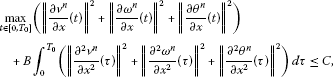(103)
$\frac{a}{2}\le {r}^{n}\left(x,t\right)\le 2M,\phantom{\rule{2em}{0ex}}\frac{{a}_{1}}{2}\le \frac{\partial {r}^{n}}{\partial x}\left(x,t\right)\le 2{M}_{1},$
(104)
$\frac{m}{2}\le {\rho }^{n}\left(x,t\right)\le 2M,\phantom{\rule{1em}{0ex}}\left(x,t\right)\in {\overline{Q}}_{0},{Q}_{0}={Q}_{{T}_{0}},$
(105)
$\underset{t\in \left[0,{T}_{0}\right]}{max}\parallel \frac{\partial {\rho }^{n}}{\partial x}\left(t\right)\parallel \le C,$
(106)
$\underset{t\in \left[0,{T}_{0}\right]}{max}\parallel \frac{{\partial }^{2}{r}^{n}}{\partial {x}^{2}}\left(t\right)\parallel \le C$
(107)

(a, ${a}_{1}$, m and M are defined by (41) and (53)-(55)).

Proof To get the estimate (103) we use an approach similar to that in  (Lemma 5.6) and  (pp.64-67). First, we introduce the function

${y}_{n}\left(t\right)={\parallel \frac{\partial {v}^{n}}{\partial x}\left(t\right)\parallel }^{2}+{\parallel \frac{\partial {\omega }^{n}}{\partial x}\left(t\right)\parallel }^{2}+{\parallel \frac{\partial {\theta }^{n}}{\partial x}\left(t\right)\parallel }^{2}+B{\int }_{0}^{t}{\parallel \frac{{\partial }^{2}{v}^{n}}{\partial {x}^{2}}\left(\tau \right)\parallel }^{2}\phantom{\rule{0.2em}{0ex}}d\tau .$
(108)

Using Lemma 4.6, we find that the function ${y}_{n}$ satisfies the differential inequality

${\stackrel{˙}{y}}_{n}\left(t\right)\le C\left(1+{y}_{n}^{8}\left(t\right)\right).$
(109)

There exists a constant $\overline{C}$ such that

$\overline{C}={\parallel \frac{d{v}_{0}}{dx}\parallel }^{2}+{\parallel \frac{d{\omega }_{0}}{dx}\parallel }^{2}+{\parallel \frac{d{\theta }_{0}}{dx}\parallel }^{2},$

and we can conclude that

${y}_{n}\left(0\right)={\parallel \frac{d{v}_{0}^{n}}{dx}\parallel }^{2}+{\parallel \frac{d{\omega }_{0}^{n}}{dx}\parallel }^{2}+{\parallel \frac{d{\theta }_{0}^{n}}{dx}\parallel }^{2}\le \overline{C}.$
(110)

We compare the solution of the problem (109)-(110) with the solution of the Cauchy problem

$\stackrel{˙}{y}\left(t\right)=C\left(1+{y}^{8}\left(t\right)\right),$
(111)
$y\left(0\right)=\overline{C}.$
(112)

Let $\left[0,{T}^{\prime }\left[$, $0<{T}^{\prime }\le T$ be an existence interval of the solution of the problem (111)-(112). From (109)-(112) we conclude that

${y}_{n}\left(t\right)\le y\left(t\right),\phantom{\rule{1em}{0ex}}t\in \left[0,{T}^{\prime }\left[.$
(113)

Let ${T}_{0}$ be such that $0<{T}_{0}\le {T}^{\prime }$. From (113) and (108) we obtain

$\underset{t\in \left[0,{T}_{0}\right]}{max}\left({\parallel \frac{\partial {v}^{n}}{\partial x}\left(t\right)\parallel }^{2}+{\parallel \frac{\partial {\omega }^{n}}{\partial x}\left(t\right)\parallel }^{2}+{\parallel \frac{\partial {\theta }^{n}}{\partial x}\left(t\right)\parallel }^{2}\right)+{\int }_{0}^{{T}_{0}}{\parallel \frac{{\partial }^{2}{v}^{n}}{\partial {x}^{2}}\left(\tau \right)\parallel }^{2}\phantom{\rule{0.2em}{0ex}}d\tau \le C$
(114)

and from (101) it follows

$\begin{array}{r}\frac{d}{dt}\left({\parallel \frac{\partial {v}^{n}}{\partial x}\left(t\right)\parallel }^{2}+{\parallel \frac{\partial {\omega }^{n}}{\partial x}\left(t\right)\parallel }^{2}+{\parallel \frac{\partial {\theta }^{n}}{\partial x}\left(t\right)\parallel }^{2}\right)\\ \phantom{\rule{1em}{0ex}}+B\left({\parallel \frac{{\partial }^{2}{v}^{n}}{\partial {x}^{2}}\left(t\right)\parallel }^{2}+{\parallel \frac{{\partial }^{2}{\omega }^{n}}{\partial {x}^{2}}\left(t\right)\parallel }^{2}+{\parallel \frac{{\partial }^{2}{\theta }^{n}}{\partial {x}^{2}}\left(t\right)\parallel }^{2}\right)\le C.\end{array}$
(115)

Integrating (101) over $\left[0,t\right]$, $0 and using estimates (110) and (115), we immediately get (103).

Now, using the inequalities (97) for the function v, we easily get

$|{v}^{n}\left(x,t\right)|\le 2\parallel \frac{\partial {v}^{n}}{\partial x}\left(t\right)\parallel ,\phantom{\rule{2em}{0ex}}\parallel \frac{\partial {v}^{n}}{\partial x}\left(t\right)\parallel \le 2\parallel \frac{{\partial }^{2}{v}^{n}}{\partial {x}^{2}}\left(t\right)\parallel ,\phantom{\rule{2em}{0ex}}|\frac{\partial {v}^{n}}{\partial x}\left(t\right)|\le 2\parallel \frac{{\partial }^{2}{v}^{n}}{\partial {x}^{2}}\left(t\right)\parallel .$
(116)

Using (116), we derive the following estimates:

${\int }_{0}^{{T}_{0}}|{v}^{n}\left(x,t\right)|\phantom{\rule{0.2em}{0ex}}d\tau \le 4{\int }_{0}^{{T}_{0}}\parallel \frac{{\partial }^{2}{v}^{n}}{\partial {x}^{2}}\left(t\right)\parallel \phantom{\rule{0.2em}{0ex}}d\tau \le 4{\left(C{B}^{-1}\right)}^{\frac{1}{2}}{T}_{0}^{\frac{1}{2}},$
(117)
${\int }_{0}^{{T}_{0}}|\frac{\partial {v}^{n}}{\partial x}\left(t\right)|\phantom{\rule{0.2em}{0ex}}d\tau \le 2{\int }_{0}^{{T}_{0}}\parallel \frac{{\partial }^{2}{v}^{n}}{\partial {x}^{2}}\left(t\right)\parallel \phantom{\rule{0.2em}{0ex}}d\tau \le 2{\left(C{B}^{-1}\right)}^{\frac{1}{2}}{T}_{0}^{\frac{1}{2}},$
(118)

where C and B are from (103). Assuming that

${T}_{0}

and using (117) and (118) from (58) and (60), we get (104)-(105).

Because of (57), (62) and (63), from (103) and (98), we easily get that for $t\in \left[0,{T}_{0}\right]$ hold

$\sum _{i=1}^{n}\left(|{v}_{i}^{n}\left(t\right)|+|{\omega }_{i}^{n}\left(t\right)|+|{\theta }_{i}^{n}\left(t\right)|\right)\le C,$
(119)
$|{\theta }_{0}^{n}\left(t\right)|\le C,$
(120)

and we can conclude that the solution of the problem (79)-(86) is defined on $\left[0,{T}_{0}\right]$. □

From (119) and (120), we can easily conclude that

$\underset{t\in \left[0,{T}_{0}\right]}{max}\left({\parallel {v}^{n}\left(t\right)\parallel }^{2}+{\parallel {\omega }^{n}\left(t\right)\parallel }^{2}+{\parallel {\theta }^{n}\left(t\right)\parallel }^{2}\right)\le C,$
(121)

and from (95), (100) and (99) it follows

$\underset{t\in \left[0,{T}_{0}\right]}{max}\parallel \frac{{\partial }^{2}{r}^{n}}{\partial {x}^{2}}\left(t\right)\parallel \le C,\phantom{\rule{2em}{0ex}}\underset{t\in \left[0,{T}_{0}\right]}{max}\parallel \frac{{\partial }^{2}{\rho }^{n}}{\partial {x}^{2}}\left(t\right)\parallel \le C,\phantom{\rule{2em}{0ex}}\underset{\left(x,t\right)\in {\overline{Q}}_{0}}{max}|{\theta }^{n}\left(t\right)|\le C.$
(122)

Lemma 4.8 Let ${T}_{0}$ be defined by Lemma  4.7. Then for each $n\in \mathbf{N}$ the inequalities

${\int }_{0}^{{T}_{0}}\left({\parallel \frac{\partial {v}^{n}}{\partial t}\left(\tau \right)\parallel }^{2}+{\parallel \frac{\partial {\omega }^{n}}{\partial t}\left(\tau \right)\parallel }^{2}+{\parallel \frac{\partial {\theta }^{n}}{\partial t}\left(\tau \right)\parallel }^{2}\right)\phantom{\rule{0.2em}{0ex}}d\tau \le C,$
(123)
$\underset{t\in \left[0,{T}_{0}\right]}{max}\parallel \frac{\partial {\rho }^{n}}{\partial t}\left(t\right)\parallel \le C,$
(124)
$\underset{t\in \left[0,{T}_{0}\right]}{max}\parallel \frac{\partial {r}^{n}}{\partial t}\left(t\right)\parallel \le C,\phantom{\rule{2em}{0ex}}\underset{t\in \left[0,{T}_{0}\right]}{max}\parallel \frac{{\partial }^{2}{r}^{n}}{\partial x\phantom{\rule{0.2em}{0ex}}\partial t}\left(t\right)\parallel \le C,\phantom{\rule{2em}{0ex}}{\int }_{0}^{{T}_{0}}{\parallel \frac{{\partial }^{2}{r}^{n}}{\partial {t}^{2}}\left(\tau \right)\parallel }^{2}\phantom{\rule{0.2em}{0ex}}d\tau \le C$
(125)

hold true.

Proof Multiplying (65) by $\frac{d{v}_{i}^{n}}{dt}\left(t\right)$, summing over $i=1,2,\dots ,n$ and using (104)-(105), we obtain

$\begin{array}{rl}{\parallel \frac{\partial {v}^{n}}{\partial t}\left(t\right)\parallel }^{2}=& -\frac{R}{L}{\int }_{0}^{1}{\left({r}^{n}\right)}^{2}\frac{\partial }{\partial x}\left({\rho }^{n}{\theta }^{n}\right)\frac{\partial {v}^{n}}{\partial t}\phantom{\rule{0.2em}{0ex}}dx\\ +\frac{\lambda +2\mu }{{L}^{2}}{\int }_{0}^{1}{\left({r}^{n}\right)}^{2}\frac{\partial }{\partial x}\left({\rho }^{n}\frac{\partial }{\partial x}\left({\left({r}^{n}\right)}^{2}{v}^{n}\right)\right)\frac{\partial {v}^{n}}{\partial t}\phantom{\rule{0.2em}{0ex}}dx\\ \le & C\left(\underset{\left(x,t\right)\in {\overline{Q}}_{0}}{max}|{\theta }^{n}\left(x,t\right)|\parallel \frac{\partial {\rho }^{n}}{\partial x}\left(t\right)\parallel \parallel \frac{\partial {v}^{n}}{\partial t}\left(t\right)\parallel +\parallel \frac{\partial {\theta }^{n}}{\partial x}\left(t\right)\parallel \parallel \frac{\partial {v}^{n}}{\partial t}\left(t\right)\parallel \\ +\underset{\left(x,t\right)\in {\overline{Q}}_{0}}{max}|{v}^{n}\left(x,t\right)|\parallel \frac{\partial {\rho }^{n}}{\partial x}\left(t\right)\parallel \parallel \frac{\partial {v}^{n}}{\partial t}\left(t\right)\parallel +\parallel {v}^{n}\left(t\right)\parallel \parallel \frac{\partial {v}^{n}}{\partial t}\left(t\right)\parallel \\ +\underset{\left(x,t\right)\in {\overline{Q}}_{0}}{max}|{v}^{n}\left(x,t\right)|\parallel \frac{{\partial }^{2}{r}^{n}}{\partial {x}^{2}}\left(t\right)\parallel \parallel \frac{\partial {v}^{n}}{\partial t}\left(t\right)\parallel +\parallel \frac{\partial {v}^{n}}{\partial t}\left(t\right)\parallel \parallel \frac{\partial {v}^{n}}{\partial x}\left(t\right)\parallel \\ +\underset{\left(x,t\right)\in {\overline{Q}}_{0}}{max}|\frac{\partial {v}^{n}}{\partial x}\left(x,t\right)|\parallel \frac{\partial {\rho }^{n}}{\partial x}\left(t\right)\parallel \parallel \frac{\partial {v}^{n}}{\partial t}\left(t\right)\parallel +\parallel \frac{{\partial }^{2}{v}^{n}}{\partial {x}^{2}}\left(t\right)\parallel \parallel \frac{\partial {v}^{n}}{\partial x}\left(t\right)\parallel \right).\end{array}$
(126)

Using (121), (122), (103), (116) and applying the Young inequality, we get

${\parallel \frac{\partial {v}^{n}}{\partial t}\left(t\right)\parallel }^{2}\le C\left(1+{\parallel \frac{{\partial }^{2}{v}^{n}}{\partial {x}^{2}}\parallel }^{2}\right)+\epsilon {\parallel \frac{\partial {v}^{n}}{\partial t}\left(t\right)\parallel }^{2}.$
(127)

Taking into account (103) for sufficiently small $\epsilon >0$ from (127), we obtain

${\int }_{0}^{{T}_{0}}{\parallel \frac{\partial {v}^{n}}{\partial t}\left(\tau \right)\parallel }^{2}\phantom{\rule{0.2em}{0ex}}d\tau \le C.$
(128)

In the same way, from (66) and (67) we obtain the estimates for $\parallel \frac{\partial {\omega }^{n}}{\partial t}\parallel$ and $\parallel \frac{\partial {\theta }^{n}}{\partial t}\parallel$ respectively. The estimates (124) and (125) follow directly from (59) and (58). □

From Lemmas 4.7 and 4.8 we easily derive the following statements.

Proposition 4.1 Let ${T}_{0}$ be defined by Lemma  4.7. Then for the sequence $\left\{\left({r}^{n},{\rho }^{n},{v}^{n},{\omega }^{n},{\theta }^{n}\right):n\in \mathbf{N}\right\}$ the following properties are satisfied:

1. (i)

$\left\{{r}^{n}\right\}$ is bounded in ${L}^{\mathrm{\infty }}\left({Q}_{0}\right)$, ${L}^{\mathrm{\infty }}\left(0,{T}_{0};{H}^{2}\left(\right]0,1\left[\right)\right)$ and ${H}^{2}\left({Q}_{0}\right)$;

2. (ii)

$\left\{\frac{\partial {r}^{n}}{\partial x}\right\}$ is bounded in ${L}^{\mathrm{\infty }}\left({Q}_{0}\right)$;

3. (iii)

$\left\{{\rho }^{n}\right\}$ is bounded in ${L}^{\mathrm{\infty }}\left({Q}_{0}\right)$, ${L}^{\mathrm{\infty }}\left(0,{T}_{0};{H}^{1}\left(\right]0,1\left[\right)\right)$ and ${H}^{1}\left({Q}_{0}\right)$;

4. (iv)

$\left\{{v}^{n}\right\}$, $\left\{{\omega }^{n}\right\}$, $\left\{{\theta }^{n}\right\}$ are bounded in ${L}^{\mathrm{\infty }}\left(0,{T}_{0};{H}^{1}\left(\right]0,1\left[\right)\right)$, ${H}^{1}\left({Q}_{0}\right)$ and ${L}^{2}\left(0,{T}_{0};{H}^{2}\left(\right]0,1\left[\right)\right)$.

## 5 The proof of Theorem 2.1

In the proofs we use some well-known facts of functional analysis (e.g., ). Let ${T}_{0}\in {\mathbf{R}}^{+}$ be defined by Lemma 4.7. Theorem 2.1 is a consequence of the following lemmas.

Lemma 5.1 There exists a function

$r\in {L}^{\mathrm{\infty }}\left(0,{T}_{0};{H}^{2}\left(\right]0,1\left[\right)\right)\cap {H}^{2}\left({Q}_{0}\right)\cap C\left({\overline{Q}}_{0}\right)$
(129)

and the subsequence of $\left\{{r}^{n}\right\}$ (for simplicity reasons denoted again as $\left\{{r}^{n}\right\}$) such that

(130)
(131)
(132)
(133)

The function r satisfies the conditions

(134)
$r\left(x,0\right)={r}_{0}\left(x\right),\phantom{\rule{1em}{0ex}}x\in \left[0,1\right],$
(135)

where ${r}_{0}$ is defined by (40).

Proof The conclusions (130) and (131) follow immediately from Proposition 4.1. Let $\left(x,t\right)$, $\left({x}^{\prime },{t}^{\prime }\right)$ belong to ${\overline{Q}}_{0}$. Then we have

$\begin{array}{r}|{r}^{n}\left(x,t\right)-{r}^{n}\left({x}^{\prime },{t}^{\prime }\right)|\le |{r}^{n}\left(x,t\right)-{r}^{n}\left({x}^{\prime },t\right)|+|{r}^{n}\left({x}^{\prime },t\right)-{r}^{n}\left({x}^{\prime },{t}^{\prime }\right)|,\\ |\frac{\partial {r}^{n}}{\partial x}\left(x,t\right)-\frac{\partial {r}^{n}}{\partial x}\left({x}^{\prime },{t}^{\prime }\right)|\le |\frac{\partial {r}^{n}}{\partial x}\left(x,t\right)-\frac{\partial {r}^{n}}{\partial x}\left({x}^{\prime },t\right)|+|\frac{\partial {r}^{n}}{\partial x}\left({x}^{\prime },t\right)-\frac{\partial {r}^{n}}{\partial x}\left({x}^{\prime },{t}^{\prime }\right)|.\end{array}$

Using (104), (58), (116), (103) and (107), we obtain

$|{r}^{n}\left(x,t\right)-{r}^{n}\left({x}^{\prime },t\right)|\le {\int }_{{x}^{\prime }}^{x}|\frac{\partial {r}^{n}}{\partial x}\left(\xi ,t\right)|\phantom{\rule{0.2em}{0ex}}d\xi \le C|x-{x}^{\prime }|,$
(136)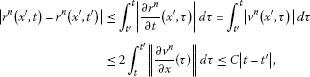(137)
$|\frac{\partial {r}^{n}}{\partial x}\left({x}^{\prime },t\right)-\frac{\partial {r}^{n}}{\partial x}\left({x}^{\prime },t\right)|\le \parallel \frac{{\partial }^{2}{r}^{n}}{\partial {x}^{2}}\left(t\right)\parallel {|x-{x}^{\prime }|}^{1/2}\le C{|x-{x}^{\prime }|}^{1/2},$
(138)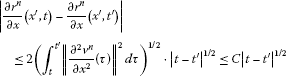(139)

and we can conclude that the sequences $\left\{{r}^{n}\right\}$ and $\left\{\frac{\partial {r}^{n}}{\partial x}\right\}$ satisfy the conditions of Arzelà-Ascoli theorem. Applying that theorem, we get the strong convergence in (132) and (133). Because of (132) and (104) we have

$\frac{a}{2}-\epsilon <{r}^{n}\left(x,t\right)-\epsilon
(140)

for each $\epsilon >0$ and sufficiently big $n\in \mathbf{N}$. From (140) we can easily conclude that (134) is satisfied. From (132) it follows

$\underset{n\to \mathrm{\infty }}{lim}\underset{x\in \left[0,1\right]}{max}|{r}^{n}\left(x,0\right)-r\left(x,0\right)|=\underset{n\to \mathrm{\infty }}{lim}\underset{x\in \left[0,1\right]}{max}|{r}_{0}\left(x\right)-r\left(x,0\right)|=0$
(141)

and because of that we have (135). □

Lemma 5.2 There exists a function

$\rho \in {L}^{\mathrm{\infty }}\left(0,{T}_{0};{H}^{1}\left(\right]0,1\left[\right)\right)\cap {H}^{1}\left({Q}_{0}\right)\cap C\left({\overline{Q}}_{0}\right)$
(142)

and the subsequence of $\left\{{\rho }^{n}\right\}$ (denoted again as $\left\{{\rho }^{n}\right\}$) such that

(143)
(144)
(145)

The function ρ satisfies the conditions

(146)
$\rho \left(x,0\right)={\rho }_{0}\left(x\right),\phantom{\rule{1em}{0ex}}x\in \left[0,1\right].$
(147)

Proof Taking into account Proposition 4.1, estimates (103)-(106) and the Arzelà-Ascoli theorem, we prove in the same way as in the previous lemma the properties (143)-(147). □

Lemma 5.3 There exist functions

$v,\omega ,\theta \in {L}^{\mathrm{\infty }}\left(0,{T}_{0};{H}^{1}\left(\right]0,1\left[\right)\right)\cap {H}^{1}\left({Q}_{0}\right)\cap {L}^{2}\left(0,{T}_{0};{H}^{2}\left(\right]0,1\left[\right)\right)$

and the subsequence of $\left\{{v}^{n},{\omega }^{n},{\theta }^{n}\right\}$ (denoted again as $\left\{{v}^{n},{\omega }^{n},{\theta }^{n}\right\}$) such that

(148)
(149)
(150)
(151)

The functions v, ω and θ satisfy the conditions

$v\left(0,t\right)=v\left(1,t\right)=\omega \left(0,t\right)=\omega \left(1,t\right)=0,\phantom{\rule{1em}{0ex}}t\in \left[0,{T}_{0}\right],$
(152)
(153)
$v\left(x,0\right)={v}_{0}\left(x\right),\phantom{\rule{2em}{0ex}}\omega \left(x,0\right)={\omega }_{0}\left(x\right)=0,\phantom{\rule{2em}{0ex}}\theta \left(x,0\right)={\theta }_{0}\left(x\right),\phantom{\rule{1em}{0ex}}x\in \left[0,1\right].$
(154)

Proof The conclusions (148)-(151) follow from Proposition 4.1 and embedding properties (see Remark 2.1). For verification of the boundary and initial conditions (152), (153) and (154), we use the Green formula as follows.

Let φ be a function of ${C}^{\mathrm{\infty }}\left(\left[0,{T}_{0}\right]\right)$ equal to zero in a neighborhood of ${T}_{0}$, with $\phi \left(0\right)\ne 0$ and $u\in {H}^{1}\left(\right]0,1\left[\right)$. Using the integration by parts we have for ${v}^{n}$ and v (e.g.) the following equalities:

$\begin{array}{r}{\int }_{0}^{{T}_{0}}{\int }_{0}^{1}\frac{\partial {v}^{n}}{\partial t}\left(x,t\right)u\left(x\right)\phi \left(t\right)\phantom{\rule{0.2em}{0ex}}dx\phantom{\rule{0.2em}{0ex}}dt+{\int }_{0}^{{T}_{0}}{\int }_{0}^{1}{v}^{n}\left(x,t\right)u\left(x\right)\frac{d\phi }{dt}\left(t\right)\phantom{\rule{0.2em}{0ex}}dx\phantom{\rule{0.2em}{0ex}}dt\\ \phantom{\rule{1em}{0ex}}=-\phi \left(0\right){\int }_{0}^{1}{v}_{0}^{n}\left(x\right)u\left(x\right)\phantom{\rule{0.2em}{0ex}}dx,\end{array}$
(155)
$\begin{array}{r}{\int }_{0}^{{T}_{0}}{\int }_{0}^{1}\frac{\partial v}{\partial t}\left(x,t\right)u\left(x\right)\phi \left(t\right)\phantom{\rule{0.2em}{0ex}}dx\phantom{\rule{0.2em}{0ex}}dt+{\int }_{0}^{{T}_{0}}{\int }_{0}^{1}v\left(x,t\right)u\left(x\right)\frac{d\phi }{dt}\left(t\right)\phantom{\rule{0.2em}{0ex}}dx\phantom{\rule{0.2em}{0ex}}dt\\ \phantom{\rule{1em}{0ex}}=-\phi \left(0\right){\int }_{0}^{1}v\left(x,0\right)u\left(x\right)\phantom{\rule{0.2em}{0ex}}dx.\end{array}$
(156)

Passing $n\to \mathrm{\infty }$ in (155) and comparing (155) and (156), we obtain

${\int }_{0}^{1}v\left(x,0\right)u\left(x\right)\phantom{\rule{0.2em}{0ex}}dx={\int }_{0}^{1}{v}_{0}\left(x\right)u\left(x\right)\phantom{\rule{0.2em}{0ex}}dx,\phantom{\rule{1em}{0ex}}\mathrm{\forall }u\in {H}^{1}\left(\right]0,1\left[\right)$
(157)

and conclude

$v\left(x,0\right)={v}_{0}\left(x\right),\phantom{\rule{1em}{0ex}}x\in \left[0,1\right].$
(158)

In the similar way, we get all the remaining properties in (152)-(154). □

Lemma 5.4 The functions r, ρ, v, ω, θ, defined by Lemmas  5.1, 5.2 and  5.3 satisfy the Equations (30)-(33) a.e. in ${Q}_{0}$.

Proof Let $\left\{\left({r}^{n},{\rho }^{n},{v}^{n},{\omega }^{n},{\theta }^{n}\right):n\in \mathbf{N}\right\}$ be subsequence defined by Lemmas 5.1, 5.2 and 5.3. Taking into account (144), (149) and strong convergencies (132), (133), (145) and (151) we get that (30) follows immediately from (59). We can write Equation (65) in the form

$\begin{array}{r}{\int }_{0}^{{T}_{0}}{\int }_{0}^{1}\frac{\partial {v}^{n}}{\partial t}\left(x,t\right)sin\left(\pi ix\right)\phi \left(t\right)\phantom{\rule{0.2em}{0ex}}dx\phantom{\rule{0.2em}{0ex}}dt\\ \phantom{\rule{1em}{0ex}}-\frac{R}{L}{\int }_{0}^{{T}_{0}}{\int }_{0}^{1}\left[{\left({r}^{n}\right)}^{2}\frac{\partial {\rho }^{n}}{\partial x}{\theta }^{n}+{\left({r}^{n}\right)}^{2}{\rho }^{n}\frac{\partial {\theta }^{n}}{\partial x}\right]sin\left(\pi ix\right)\phi \left(t\right)\phantom{\rule{0.2em}{0ex}}dx\phantom{\rule{0.2em}{0ex}}dt\\ \phantom{\rule{1em}{0ex}}-\frac{\lambda +2\mu }{{L}^{2}}{\int }_{0}^{{T}_{0}}{\int }_{0}^{1}\left[2{\left({r}^{n}\right)}^{3}\frac{\partial {\rho }^{n}}{\partial x}\frac{\partial {r}^{n}}{\partial x}{v}^{n}+2{\left({r}^{n}\right)}^{2}{\rho }^{n}{\left(\frac{\partial {r}^{n}}{\partial x}\right)}^{2}{v}^{n}\\ \phantom{\rule{1em}{0ex}}+2{\left({r}^{n}\right)}^{3}{\rho }^{n}\frac{{\partial }^{2}{r}^{n}}{\partial {x}^{2}}{v}^{n}+4{\left({r}^{n}\right)}^{3}{\rho }^{n}\frac{\partial {r}^{n}}{\partial x}\frac{\partial {v}^{n}}{\partial x}\\ \phantom{\rule{1em}{0ex}}+{\left({r}^{n}\right)}^{4}\frac{\partial {\rho }^{n}}{\partial x}\frac{\partial {v}^{n}}{\partial x}+{\left({r}^{n}\right)}^{4}{\rho }^{n}\frac{{\partial }^{2}{v}^{n}}{\partial {x}^{2}}\right]sin\left(\pi ix\right)\phi \left(t\right)\phantom{\rule{0.2em}{0ex}}dx\phantom{\rule{0.2em}{0ex}}dt=0\end{array}$
(159)

for each $\phi \in \mathfrak{D}\left(\right]0,{T}_{0}\left[\right)$, where $\mathfrak{D}$ denotes the space of test functions. Now we consider the convergence of one integrand. For example, we will prove the convergence

${\int }_{0}^{{T}_{0}}{\int }_{0}^{1}{\left({r}^{n}\right)}^{3}{\rho }^{n}\frac{{\partial }^{2}{r}^{n}}{\partial {x}^{2}}{v}^{n}sin\left(\pi ix\right)\phi \left(t\right)\phantom{\rule{0.2em}{0ex}}dx\phantom{\rule{0.2em}{0ex}}dt\to {\int }_{0}^{{T}_{0}}{r}^{3}\rho \frac{{\partial }^{2}r}{\partial {x}^{2}}vsin\left(\pi ix\right)\phi$